# Introduction

This class covers Python from the very basics. Suitable for GIS practitioners with no programming background or python knowledge. The course will introduce participants to basic programming concepts, libraries for spatial analysis, geospatial APIs and techniques for building spatial data processing pipelines.

# Get the Data Package

The code examples in this class use a variety of datasets. All the required datasets and Jupyter notebooks are supplied to you in the python_foundation.zip file. Unzip this file to a directory - preferably to the <home folder>/Downloads/python_foundation/ folder.

Not enrolled in our instructor-led class but want to work through the material on your own? Get free access to the data package

# Installation and Setting up the Environment

There are many ways to install Python on your system. Many operating systems comes with a Python distribution built-in too. If you use software such as QGIS - it comes with its own version of Python. Working on Python projects typically require installing third-party packages (i.e. tools). As these packages have different dependencies - they often cause conflicts and errors due to different versions of Python on your system.

An easy and reliable way to get a Python installation on your system is via Anaconda. For this course, we will use the Anaconda3 Individual Edition to install Python and required spatial analysis packages.

Many Python tool names have references to the reptile world. The default Python package manager is called Pip which references the process of hatching eggs. Interestingly, the naming of the Python language itself had nothing to do with reptiles.

Anaconda provides the conda command that can be run from the Command Prompt on Windows or the Terminal on Mac/Linux. This is the preferred method to install the required packages.

1. Download the Anaconda Installer for Python 3.8 (or a higher version) for your operating system. Once downloaded, double click the installer and install it into the default suggested directory. Select an install for Just Me and use default settings.

Note: If your username has spaces, or non-English characters, it causes problems. In that case, you can install it to a path such as C:\anaconda.1. (Windows users) Once installed, search for Anaconda Prompt launch it. (Mac/Linux users): Launch a Terminal window.1. It is a good practice to create a new environment for each of your Python projects. An environment is a space where you will install required packages. Many packages may contain conflicting requirements, preventing them from all being installed into a single environment. Having a separate environment isolates your project from such problems. We will now type commands in a terminal to create a new environment. (Windows users) Search for Anaconda Prompt in the Start Menu and launch it. (Mac/Linux users): Open a Terminal window. Enter the command below and press Enter to create your new environment.
conda create --name python_foundation1. Now you will be asked for confirmation; press y + Enter to proceed and complete your environment creation.1. Now that the environment is created, you need to activate it. Type the command below and press Enter. Once the environment activates, the (base) will change to (python_foundation).
conda activate python_foundation1. Now we are ready to install the required packages using the conda install command. First we will install geopandas. This is a module that allows you to work with vector geospatial data in Python. We will use the conda-forge channel to install the package. The -y option will skip the confirmation dialog. Enter the command below and press Enter.
conda install --channel conda-forge geopandas -y1. If the installation is successful, you should see a message as shown here.1. geopandas installation is a bit tricky and can sometimes fail. So it is a good idea to check if it can be imported without errors. We will use the python command with the -c option which will execute a python statement. Enter the below command and press Enter.
python -c "import geopandas"1. If your geopandas installation was successful, the command will run without any output. If nothing happens - it means your installation was successful!1. We can now install other required packages for this class. Run the command below to install geopy, rasterio, matplotlib and jupyterlab packages.
conda install --channel conda-forge geopy rasterio matplotlib jupyterlab -y1. Once the command finishes, you should see a screen such as below.1. Your Python environment is now ready. Launch the JupyterLab application using the command below. This will initiate and run a local server in your system and opens in your default browser.

Note: Do not close your anaconda prompt after JupyterLab opens up. You need to keep it running as long as you want to use JupyterLab.

jupyter-labNote that JupyterLab application can browse the files only on the Drive from where it was launched from. If your data is stored on a different drive, you will need an additional step to switch to that drive before launching Jupyterlab.

Windows

On the command prompt, type the drive letter followed by : and press Enter to switch to the drive.

D:
jupyter-labMac/Linux

Check the drives mounted on your system by entering ls /Volumes. After that use cd command to switch to the drive.

cd /Volumes/<NameofYourDrive>
jupyter-lab
1. A new browser tab will open with an instance of JupyterLab. Click the Python 3 button under Notebook.1. Enter the following statements in the first cell and click the Run button. If nothing happens - it means your installation was successful!. Your environment is now ready for the course. If you get an ImportError, repeat the installation steps carefully again.
import geopandas
import geopy
import rasterio# Using Jupyter Notebooks

Your class data package contain multiple Jupyter notebooks containing code and exercises for this class.

1. Launch the JupyterLab application. It will open your Web Browser and load the application in a new tab. From the left-hand panel, navigate to the directory where you extracted the data package.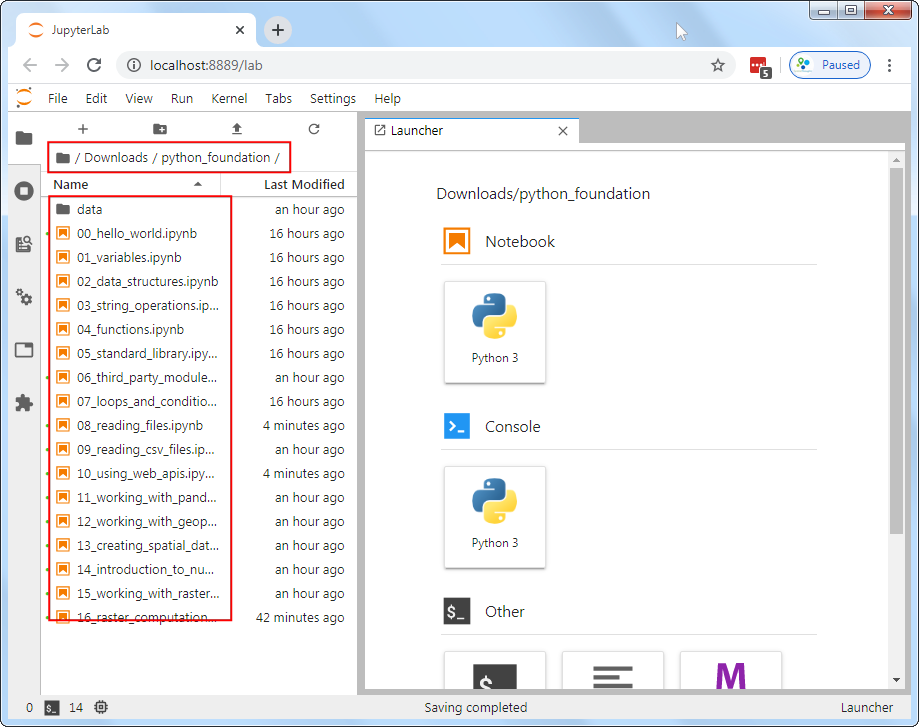1. Jupyter notebooks have a .ipynb extensions. Double-click on a notebook file to open it. Code in the notebook is executed cell-by-cell. You can select a cell and click the Run button to execute the code and see the output.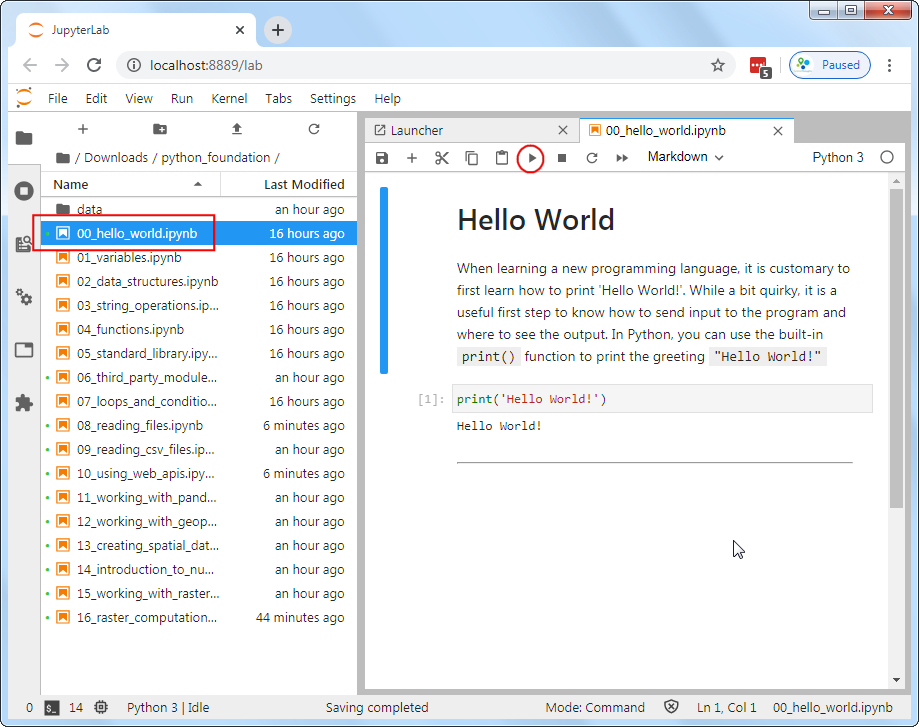1. At the end of each notebook, you will find an exercise. Before adding a new cell and attempting to complete the exercise, make sure you go to Run → Run All Cells to execute all the code in the notebook. Doing this will ensure all the required variables are avalable to you to use in the exervise.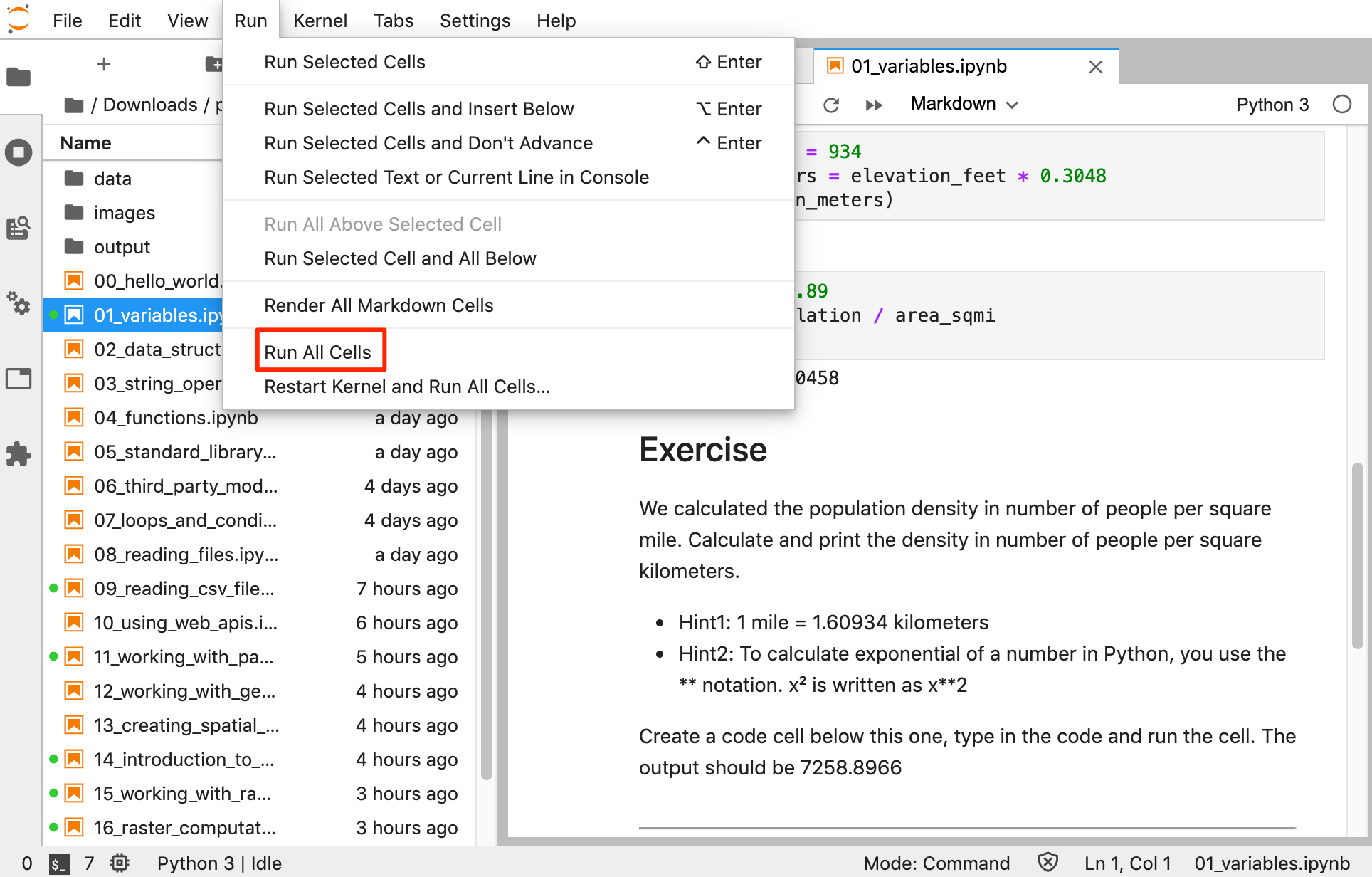Open the notebook named 00_hello_world.ipynb.

# Hello World

When learning a new programming language, it is customary to first learn how to print ‘Hello World!’. While a bit quirky, it is a useful first step to know how to send input to the program and where to see the output. In Python, you can use the built-in print() function to print the greeting "Hello World!"

print('Hello World!')

Open the notebook named 01_variables.ipynb.

# Variables

## Strings

A string is a sequence of letters, numbers, and punctuation marks - or commonly known as text

In Python you can create a string by typing letters between single or double quotation marks.

city = 'San Fransico'
state = 'California'
print(city, state)
print(city + state)
print(city + ',' + state)

## Numbers

Python can handle several types of numbers, but the two most common are:

• int, which represents integer values like 100, and
• float, which represents numbers that have a fraction part, like 0.5
population = 881549
latitude = 37.7739
longitude = -121.5687
print(type(population))
print(type(latitude))
elevation_feet = 934
elevation_meters = elevation_feet * 0.3048
print(elevation_meters)
area_sqmi = 46.89
density = population / area_sqmi
print(density)

## Exercise

We have a variable named distance_km below with the value 4135 - indicating the straight-line distance between San Francisco and New York in Kilometers. Create another variable called distance_mi and store the distance value in miles.

• Hint1: 1 mile = 1.60934 kilometers

Add the code in the cell below and run it. The output should be 2569.37

distance_km = 4135
# Remove this line and add code here

Open the notebook named 02_data_structures.ipynb.

# Data Structures

## Tuples

A tuple is a sequence of objects. It can have any number of objects inside. In Python tuples are written with round brackets ().

latitude = 37.7739
longitude = -121.5687
coordinates = (latitude, longitude)
print(coordinates)

You can access each item by its position, i.e. index. In programming, the counting starts from 0. So the first item has an index of 0, the second item an index of 1 and so now. The index has to be put inside square brackets [].

y = coordinates
x = coordinates
print(x, y)

## Lists

A list is similar to a tuple - but with a key difference. With tuples, once created, they cannot be changed, i.e. they are immutable. But lists are mutable. You can add, delete or change elements within a list. In Python, lists are written with square brackets []

cities = ['San Francisco', 'Los Angeles', 'New York', 'Atlanta']
print(cities)

You can access the elements from a list using index the same way as tuples.

print(cities)

You can call len() function with any Python object and it will calculates the size of the object.

print(len(cities))

We can add items to the list using the append() method

cities.append('Boston')
print(cities)

As lists are mutable, you will see that the size of the list has now changed

print(len(cities))

Another useful method for lists is sort() - which can sort the elements in a list.

cities.sort()
print(cities)

The default sorting is in ascending order. If we wanted to sort the list in a decending order, we can call the function with reverse=True

cities.sort(reverse=True)
print(cities)

## Sets

Sets are like lists, but with some interesting properties. Mainly that they contain only unique values. It also allows for set operations - such as intersection, union and difference. In practice, the sets are typically created from lists.

capitals = ['Sacramento', 'Boston', 'Austin', 'Atlanta']
capitals_set = set(capitals)
cities_set = set(cities)

capital_cities = capitals_set.intersection(cities_set)
print(capital_cities)

Sets are also useful in finding unique elements in a list. Let’s merge the two lists using the extend() method. The resulting list will have duplicate elements. Creating a set from the list removes the duplicate elements.

cities.extend(capitals)
print(cities)
print(set(cities))

## Dictionaries

In Python dictionaries are written with curly brackets {}. Dictionaries have keys and values. With lists, we can access each element by its index. But a dictionary makes it easy to access the element by name. Keys and values are separated by a colon :.

data = {'city': 'San Francisco', 'population': 881549, 'coordinates': (-122.4194, 37.7749) }
print(data)

You can access an item of a dictionary by referring to its key name, inside square brackets.

print(data['city'])

## Exercise

From the dictionary below, how do you access the latitude and longitude values? print the latitude and longitude of new york city by extracting it from the dictionary below.

The expected output should look like below.

40.661
-73.944
nyc_data = {'city': 'New York', 'population': 8175133, 'coordinates': (40.661, -73.944) }

Open the notebook named 03_string_operations.ipynb.

# String Operations

city = 'San Francisco'
print(len(city))
print(city.split())
print(city.upper())
city
city[-1]
city[0:3]
city[4:]

## Escaping characters

Certain characters are special since they are by Python language itself. For example, the quote character is used to define a string. What do you do if your string contains a quote character?

In Python strings, the backslash \ is a special character, also called the escape character. Prefixing any character with a backslash makes it an ordinary character. (Hint: Prefixing a backslash with a backshalsh makes it ordinary too!)

It is also used for representing certain whitespace characters, \n is a newline, \t is a tab etc.

Remove the # from the cell below and run it.

# my_string = 'It's a beautiful day!'

We can fix the error by espacing the single quote within the string.

my_string = 'It\'s a beautiful day!'
print(my_string)

Alternatively, you can also use double-quotes if your string contains a single-quote.

my_string = "It's a beautiful day!"

What if our string contains both single and double quotes?

We can use triple-quotes! Enclosing the string in triple quotes ensures both single and double quotes are treated correctly.

latitude = '''37° 46' 26.2992" N'''
longitude = '''122° 25' 52.6692" W'''
print(latitude, longitude)

Backslashes pose another problem when dealing with Windows paths

#path = 'C:\Users\ujaval'
#print(path)

Prefixing a string with r makes is a Raw string. Which doesn’t interpret backslash as a special character

path = r'C:\Users\ujaval'
print(path)

## Printing Strings

Modern way of creating strings from variables is using the format() method

city = 'San Fransico'
population = 881549
output = 'Population of {} is {}.'.format(city, population)
print(output)

You can also use the format method to control the precision of the numbers

latitude = 37.7749
longitude = -122.4194

coordinates = '{:.2f},{:.2f}'.format(latitude, longitude)
print(coordinates)

## Exercise

Use the string slicing to extract and print the degrees, minutes and second parts of the string below. The output should be as follows

37
46
26.2992
latitude = '''37° 46' 26.2992"'''

Open the notebook named 04_loops_and_conditionals.ipynb.

# Loops and Conditionals

## For Loops

A for loop is used for iterating over a sequence. The sequence can be a list, a tuple, a dictionary, a set, or a string.

cities = ['San Francisco', 'Los Angeles', 'New York', 'Atlanta']

for city in cities:
print(city)

To iterate over a dictionary, you can call the items() method on it which returns a tuple of key and value for each item.

data = {'city': 'San Francisco', 'population': 881549, 'coordinates': (-122.4194, 37.7749) }

for x, y in data.items():
print(x, y)

The built-in range() function allows you to create sequence of numbers that you can iterate over

for x in range(5):
print(x)

The range function can also take a start and an end number

for x in range(1, 10, 2):
print(x)

# Conditionals

Python supports logical conditions such as equals, not equals, greater than etc. These conditions can be used in several ways, most commonly in if statements and loops.

An if statement is written by using the if keyword.

Note: A very common error that programmers make is to use = to evaluate a equals to condition. The = in Python means assignment, not equals to. Always ensure that you use the == for an equals to condition.

for city in cities:
if city == 'Atlanta':
print(city)

You can use else keywords along with if to match elements that do not meet the condition

for city in cities:
if city == 'Atlanta':
print(city)
else:
print('This is not Atlanta')

Python relies on indentation (whitespace at the beginning of a line) to define scope in the for loop and if statements. So make sure your code is properly indented.

You can evaluate a series of conditions using the elif keyword.

Multiple criteria can be combined using the and and or keywords.

cities_population = {
'San Francisco': 881549,
'Los Angeles': 3792621,
'New York': 8175133,
'Atlanta':498044
}

for city, population in cities_population.items():
if population < 1000000:
print('{} is a small city'.format(city))
elif population > 1000000 and population < 5000000:
print('{} is a big city'.format(city))
else:
print('{} is a mega city'.format(city))

## Control Statements

A for-loop iterates over each item in the sequence. Sometimes is desirable to stop the execution, or skip certain parts of the for-loops. Python has special statements, break, continue and pass.

A break statement will stop the loop and exit out of it

for city in cities:
print(city)
if city == 'Los Angeles':
print('I found Los Angeles')
break

A continue statement will skip the remaining part of the loop and go to the next iteration

for city in cities:
if city == 'Los Angeles':
continue
print(city)

A pass statement doesn’t do anything. It is useful when some code is required to complete the syntax, but you do not want any code to execute. It is typically used as a placeholder when a function is not complete.

for city in cities:
if city == 'Los Angeles':
pass
else:
print(city)

## Exercise

The Fizz Buzz challenge.

Write a program that prints the numbers from 1 to 100 and for multiples of 3 print Fizz instead of the number and for the multiples of 5 print Buzz. If it is divisible by both, print FizzBuzz.

So the output should be something like below

1, 2, Fizz, 4, Buzz, Fizz, 7, 8, Fizz, Buzz, 11, Fizz, 13, 14, FizzBuzz, ...

Breaking down the problem further, we need to create for-loop with following conditions

• If the number is a multiple of both 3 and 5 (i.e. 15), print FizzBuzz
• If the number is multiple of 3, print Fizz
• If the number is multiple of 5, print Buzz
• Otherwise print the number

Hint: See the code cell below. Use the modulus operator % to check if a number is divisible by another. 10 % 5 equals 0, meaning it is divisible by 5.

for x in range(1, 10):
if x%2 == 0:
print('{} is divisible by 2'.format(x))
else:
print('{} is not divisible by 2'.format(x))

Open the notebook named 05_functions.ipynb.

# Functions

A function is a block of code that takes one or more inputs, does some processing on them and returns one or more outputs. The code within the function runs only when it is called.

A funtion is defined using the def keyword

def my_function():
....
....
return something

Functions are useful because they allow us to capture the logic of our code and we can run it with differnt inputs without having to write the same code again and again.

def greet(name):
return 'Hello ' + name

print(greet('World'))

Functions can take multiple arguments. Let’s write a function to convert coordinates from degrees, minutes, seconds to decimal degrees. This conversion is needed quite often when working with data collected from GPS devices.

• 1 degree is equal to 60 minutes
• 1 minute is equal to 60 seconds (3600 seconds)

To calculate decimal degrees, we can use the formula below:

If degrees are positive:

Decimal Degrees = degrees + (minutes/60) + (seconds/3600)

If degrees are negative

Decimal Degrees = degrees - (minutes/60) - (seconds/3600)

def dms_to_decimal(degrees, minutes, seconds):
if degrees < 0:
result = degrees - minutes/60 - seconds/3600
else:
result = degrees + minutes/60 + seconds/3600
return result
output = dms_to_decimal(10, 10, 10)
print(output)

## Exercise

Given a coordinate string with value in degree, minutes and seconds, convert it to decimal degrees by calling the dms_to_decimal function.

def dms_to_decimal(degrees, minutes, seconds):
if degrees < 0:
result = degrees - minutes/60 - seconds/3600
else:
result = degrees + minutes/60 + seconds/3600
return result

coordinate = '''37° 46' 26.2992"'''

# Add the code below to extract the parts from the coordinate string,
# call the function to convert to decimal degrees and print the result
# The expected answer is 37.773972
# Hint: Converting strings to numbers
# When you extract the parts from the coordinate string, they are strings
# You will need to use the built-in int() / float() functions to
# convert them to numbers
x = '25'
print(x, type(x))
y = int(x)
print(y, type(y))

Open the notebook named 06_standard_library.ipynb.

# The Python Standard Library

Python comes with many built-in modules that offer ready-to-use solutions to common programming problems. To use these modules, you must use the import keyword. Once imported in your Python script, you can use the functions provided by the module in your script.

We will use the built-in math module that allows us to use advanced mathematical functions.

import math

You can also import specific functions or constants from the module like below

from math import pi
print(pi)

## Calculating Distance

Given 2 points with their Latitude and Longitude coordinates, the Haversine Formula calculates the straight-line distance in meters, assuming that Earth is a sphere.

The formula is simple enough to be implemented in a spreadsheet too. If you are curious, see my post about using this formula for calculating distances in a spreadsheet.

We can write a function that accepts a pair of origin and destination coordinates and computes the distance.

san_francisco = (37.7749, -122.4194)
new_york = (40.661, -73.944)
def haversine_distance(origin, destination):
lat1, lon1 = origin
lat2, lon2 = destination
a = math.sin(dlat/2) * math.sin(dlat/2) + math.cos(math.radians(lat1)) \
* math.cos(math.radians(lat2)) * math.sin(dlon/2) * math.sin(dlon/2)
c = 2 * math.atan2(math.sqrt(a), math.sqrt(1-a))
return distance
distance = haversine_distance(san_francisco, new_york)
print(distance/1000, 'km')

## Discover Python Easter Eggs

Programmers love to hide secret jokes in their programs for gun. These are known as Easter Eggs. Python has an easter egg that you can see when you try to import the module named this. Try writing the command import this below.

import this

Let’s try one more. Try importing the antigravity module.

Here’s a complete list of easter eggs in Python.

## Exercise

Find the coordinates of 2 cities near you and calculate the distance between them by calling the haversine_distance function below.

def haversine_distance(origin, destination):
lat1, lon1 = origin
lat2, lon2 = destination
a = math.sin(dlat/2) * math.sin(dlat/2) + math.cos(math.radians(lat1)) \
* math.cos(math.radians(lat2)) * math.sin(dlon/2) * math.sin(dlon/2)
c = 2 * math.atan2(math.sqrt(a), math.sqrt(1-a))
return distance

# city1 = (lat1, lng1)
# city2 = (lat2, lng2)
# call the function and print the result

Open the notebook named 07_third_party_modules.ipynb.

# Third-party Modules

Python has a thriving ecosystem of third-party modules (i.e. libraries or packages) available for you to install. There are hundreds of thousands of such modules available for you to install and use.

## Installing third-party libraries

Python comes with a package manager called pip. It can install all the packages listed at PyPI (Python Package Index). To install a package using pip, you need to run a command like following in a Terminal or CMD prompt.

pip install <package name>

For this course, we are using Anancoda platform - which comes with its own package manager called conda. You can use Anaconda Navigator to search and install packages. Or run the command like following in a Terminal or CMD Prompt.

conda install <package name>

See this comparison of pip and conda to understand the differences.

## Calculating Distance

We have already installed the geopy package in our environment. geopy comes with functions that have already implemented many distance calculation formulae.

• distance.great_circle(): Calculates the distance on a great circle using haversine formula
• distance.geodesic(): Calculates the distance using a chosen ellipsoid using vincenty’s formula
from geopy import distance

san_francisco = (37.7749, -122.4194)
new_york = (40.661, -73.944)

straight_line_distance = distance.great_circle(san_francisco, new_york)
ellipsoid_distance = distance.geodesic(san_francisco, new_york, ellipsoid='WGS-84')

print(straight_line_distance, ellipsoid_distance)

## Exercise

Repeat the distance calculation exercise from the previous module but perform the calculation using the geopy library.

from geopy import distance

# city1 = (lat1, lng1)
# city2 = (lat2, lng2)
# call the geopy distance function and print the great circle and ellipsoid distance

Open the notebook named 08_using_web_apis.ipynb.

# Using Web APIs

An API, or Application Program Interface, allows one program to talk to another program. Many websites or services provide an API so you can query for information in an automated way.

For mapping and spatial analysis, being able to use APIs is critical. For the longest time, Google Maps API was the most popular API on the web. APIs allow you to query web servers and get results without downloading data or running computation on your machine.

Common use cases for using APIs for spatial analysis are

• Getting directions / routing
• Route optimization
• Geocoding
• Getting real-time weather data

The provide of such APIs have many ways to implement an API. There are standards such as REST, SOAP, GraphQL etc. REST is the most populat standard for web APIs, and for geospatial APIs. REST APIs are used over HTTP and thus called web APIs.

## Understanding JSON and GeoJSON

JSON stands for JavaScript Object Notation. It is a format for storing and transporting data, and is the de-facto standard for data exchanged by APIs. GeoJSON is an extension of the JSON format that is commonly used to represent spatial data.

Python has a built-in json module that has methods for reading json data and converting it to Python objects, and vice-versa. In this example, we are using the requests module for querying the API which conveniently does the conversion for us. But it is useful to learn the basics of working with JSON in Python.

The GeoJSON data contains features, where each feature has some properties and a geometry.

geojson_string = '''
{
"type": "FeatureCollection",
"features": [
{"type": "Feature",
"properties": {"name": "San Francisco"},
"geometry": {"type": "Point", "coordinates": [-121.5687, 37.7739]}
}
]
}
'''
print(geojson_string)

To convert a JSON string to a Python object (i.e. parsing JSON), we can use the json.loads() method.

import json

print(type(data))
print(data)

Now that we have parsed the GeoJSON string and have a Python object, we can extract infromation from it. The data is stored in a FeatureCollection - which is a list of features. In our example, we have just 1 feature inside the feature collection, so we can access it by using index 0.

city_data = data['features']
print(city_data)

The feature representation is a dictionary, and individual items can be accesses using the keys

city_name = city_data['properties']['name']
city_coordinates = city_data['geometry']['coordinates']
print(city_name, city_coordinates)

## The requests module

To query a server, we send a GET request with some parameters and the server sends a response back. The requests module allows you to send HTTP requests and parse the responses using Python.

The response contains the data received from the server. It contains the HTTP status_code which tells us if the request was successful. HTTP code 200 stands for Sucess OK.

import requests

response = requests.get('https://www.spatialthoughts.com')

print(response.status_code)

## Calculating Distance using OpenRouteService API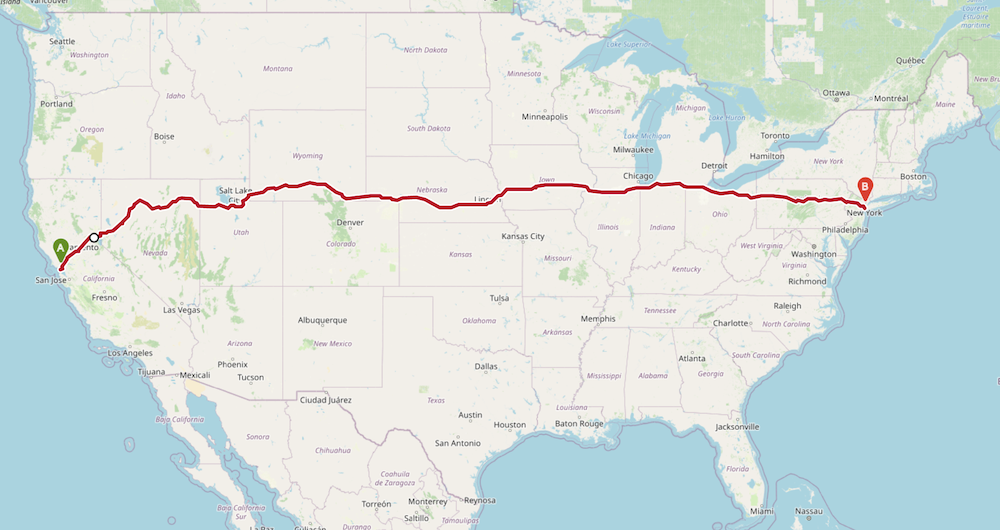OpenRouteService (ORS) provides a free API for routing, distance matrix, geocoding, route optimization etc. using OpenStreetMap data. We will learn how to use this API through Python and get real-world distance between cities.

Almost all APIs require you to sign-up and obtain a key. The key is used to identify you and enforce usage limits so that you do not overwhelm the servers. We will obtain a key from OpenRouteServie so we can use their API

Visit OpenRouteService Sign-up page and create an account. Once your account is activated, visit your Dashboard and request a token. Select Free as the Token type and enter python_foundation as the Token name. Click CREATE TOKEN. Once created, copy the long string displayed under Key and enter below.

ORS_API_KEY = '<replace this with your key>'

We will use the OpenRouteServices’s Directions Service. This service returns the driving, biking or walking directions between the given origin and destination points.

import requests

san_francisco = (37.7749, -122.4194)
new_york = (40.661, -73.944)

parameters = {
'api_key': ORS_API_KEY,
'start' : '{},{}'.format(san_francisco, san_francisco),
'end' : '{},{}'.format(new_york, new_york)
}

response = requests.get(
'https://api.openrouteservice.org/v2/directions/driving-car', params=parameters)

if response.status_code == 200:
print('Request successful.')
data = response.json()
else:
print('Request failed.')

We can read the response in JSON format by calling json() method on it.

data = response.json()

The response is a GeoJSON object representing the driving direction between the 2 points. The object is a feature collection with just 1 feature. We can access it using the index 0. The feature’s property contains summary information which has the data we need.

summary = data['features']['properties']['summary']
print(summary)

We can extract the distance and convert it to kilometers.

distance = summary['distance']
print(distance/1000)

You can compare this distance to the straight-line distance and see the difference.

## API Rate Limiting

Many web APIs enforce rate limiting - allowing a limited number of requests over time. With computers it is easy to write a for loop, or have multiple programs send hundrends or thousands of queries per second. The server may not be configured to handle such volume. So the providers specify the limits on how many and how fast the queries can be sent.

OpenRouteService lists several API Restrictions. The free plan allows for upto 40 direction requests/minute.

There are many libraries available to implement various strategies for rate limiting. But we can use the built-in time module to implement a very simple rate limiting method.

### The time module

Python Standard Library has a time module that allows for time related operation. It contains a method time.sleep() which delays the execution of the program for the specified number of seconds.

import time
for x in range(10):
print(x)
time.sleep(1)

## Exercise

Below cell contains a dictionary with 3 destination cities and their coordinates. Write a for loop to iterate over the destination_cities disctionary and call get_driving_distance() function to print real driving distance between San Fransico and each city. Rate limit your queries by adding time.sleep(2) between successive function calls.

import csv
import os
import requests
import time
ORS_API_KEY = '<replace this with your key>'

def get_driving_distance(source_coordinates, dest_coordinates):
parameters = {
'api_key': ORS_API_KEY,
'start' : '{},{}'.format(source_coordinates, source_coordinates),
'end' : '{},{}'.format(dest_coordinates, dest_coordinates)
}

response = requests.get(
'https://api.openrouteservice.org/v2/directions/driving-car', params=parameters)

if response.status_code == 200:
data = response.json()
summary = data['features']['properties']['summary']
distance = summary['distance']
return distance/1000
else:
print('Request failed.')
return -9999

san_francisco = (37.7749, -122.4194)

destination_cities = {
'Los Angeles': (34.0522, -118.2437),
'Boston': (42.3601, -71.0589),
'Atlanta': (33.7490, -84.3880)
}

Open the notebook named 09_reading_files.ipynb.

Python provides built-in functions for reading and writing files.

To read a file, we must know the path of the file on the disk. Python has a module called os that has helper functions that helps dealing with the the operating system. Advantage of using the os module is that the code you write will work without change on any suppored operating systems.

import os

To open a file, we need to know the path to the file. We will now open and read the file worldcitites.csv located in your data package. In your data package the data folder is in the data/ directory. We can construct the relative path to the file using the os.path.join() method.

data_pkg_path = 'data'
filename = 'worldcities.csv'
path = os.path.join(data_pkg_path, filename)
print(path)

To open the file, use the built-in open() function. We specify the mode as r which means read-only. If we wanted to change the file contents or write a new file, we would open it with w mode.

Our input file also contains Unicode characters, so we specify UTF-8 as the encoding.

The open() function returns a file object. We can call the readline() method for reading the content of the file, one line at a time.

It is a good practice to always close the file when you are done with it. To close the file, we must call the close() method on the file object.

f = open(path, 'r', encoding='utf-8')
f.close()

Calling readline() for each line of the file is tedious. Ideally, we want to loop through all the lines in file. You can iterate through the file object like below.

We can loop through each line of the file and increase the count variable by 1 for each iteration of the loop. At the end, the count variable’s value will be equal to the number of lines in the file.

f = open(path, 'r', encoding='utf-8')

count = 0
for line in f:
count += 1
f.close()
print(count)

## Exercise

Print first 5 lines of the file.

• Hint: Use break statement
import os
data_pkg_path = 'data'
filename = 'worldcities.csv'
path = os.path.join(data_pkg_path, filename)

# Add code to open the file and read first 5 lines

Open the notebook named 10_reading_csv_files.ipynb.

Comma-separated Values (CSV) are the most common text-based file format for sharing geospatial data. The structure of the file is 1 data record per line, with individual columns separated by a comma.

In general, the separator character is called a delimiter. Other popular delimiters include the tab (\t), colon (:) and semi-colon (;) characters.

Reading CSV file properly requires us to know which delimiter is being used, along with quote character to surround the field values that contain space of the delimiter character. Since reading delimited text file is a very common operation, and can be tricky to handle all the corner cases, Python comes with its own library called csv for easy reading and writing of CSV files. To use it, you just have to import it.

import csv

The preferred way to read CSV files is using the DictReader() method. Which directly reads each row and creates a dictionary from it - with column names as key and column values as value. Let’s see how to read a file using the csv.DictReader() method.

import os
data_pkg_path = 'data'
filename = 'worldcities.csv'
path = os.path.join(data_pkg_path, filename)
f = open(path, 'r')
f.close()

## Using enumerate() function

When iterating over an object, many times we need a counter. We saw in the previous example, how to use a variable like count and increase it with every iteration. There is an easy way to do this using the built-in enumerate() function.

cities = ['San Francisco', 'Los Angeles', 'New York', 'Atlanta']
for x in enumerate(cities):
print(x)

We can use enumerate() on any iterable object and get a tuple with an index and the iterable value with each iteration. Let’s use it to print the first 5 lines from the DictReader object.

f = open(path, 'r', encoding='utf-8')
print(row)
if index == 4:
break
f.close()

## Using with statement

The code for file handling requires we open a file, do something with the file object and then close the file. That is tedious and it is possible that you may forget to call close() on the file. If the code for processing encounters an error the file is not closed property, it may result in bugs - especially when writing files.

The preferred way to work with file objects is using the with statement. It results in simpler and cleaer code - which also ensures file objects are closed properly in case of errors.

As you see below, we open the file and use the file object f in a with statement. Python takes care of closing the file when the execution of code within the statement is complete.

with open(path, 'r', encoding='utf-8') as f:
csv_reader = csv.DictReader(f)

## Filtering rows

We can use conditional statement while iterating over the rows, to select and process rows that meet certain criterial. Let’s count how many cities from a particular country are present in the file.

Replace the home_country variable with your home country below.

home_country = 'India'
num_cities = 0

with open(path, 'r', encoding='utf-8') as f:

if row['country'] == home_country:
num_cities += 1

print(num_cities)

## Calculating distance

Let’s apply the skills we have learnt so far to solve a complete problem. We want to read the worldcities.csv file, find all cities within a home country, calculate the distance to each cities from a home city and write the results to a new CSV file.

First we find the coordinates of the out selected home_city from the file. Replace the home_city below with your hometown or a large city within your country. Note that we are using the city_ascii field for city name comparison, so make sure the home_city variable contains the ASCII version of the city name.

home_city = 'Bengaluru'

home_city_coordinates = ()

with open(path, 'r', encoding='utf-8') as f:
if row['city_ascii'] == home_city:
lat = row['lat']
lng = row['lng']
home_city_coordinates = (lat, lng)
break

print(home_city_coordinates)

Now we can loop through the file, find a city in the chosen home country and call the geopy.distance.geodesic() function to calculate the distance. In the code below, we are just computing first 5 matches.

from geopy import distance

counter = 0
with open(path, 'r', encoding='utf-8') as f:
if (row['country'] == home_country and
row['city_ascii'] != home_city):
city_coordinates = (row['lat'], row['lng'])
city_distance = distance.geodesic(
city_coordinates, home_city_coordinates).km
print(row['city_ascii'], city_distance)
counter += 1

if counter == 5:
break


## Writing files

Instead of printing the results, let’s write the results to a new file. Similar to csv.DictReader(), there is a companion csv.DictWriter() method to write files. We create a csv_writer object and then write rows to it using the writerow() method.

First we create an output folder to save the results. We can first check if the folder exists and if it doesn’t exist, we can create it.

output_dir = 'output'
if not os.path.exists(output_dir):
os.mkdir(output_dir)
output_filename = 'cities_distance.csv'
output_path = os.path.join(output_dir, output_filename)

with open(output_path, mode='w', encoding='utf-8') as output_file:
fieldnames = ['city', 'distance_from_home']
csv_writer = csv.DictWriter(output_file, fieldnames=fieldnames)

# Now we read the input file, calculate distance and
# write a row to the output
with open(path, 'r', encoding='utf-8') as f:
if (row['country'] == home_country and
row['city_ascii'] != home_city):
city_coordinates = (row['lat'], row['lng'])
city_distance = distance.geodesic(
city_coordinates, home_city_coordinates).km
csv_writer.writerow(
{'city': row['city_ascii'],
'distance_from_home': city_distance}
)

Below is the complete code for our task of reading a file, filtering it, calculating distance and writing the results to a file.

import csv
import os
from geopy import distance

data_pkg_path = 'data'
input_filename = 'worldcities.csv'
input_path = os.path.join(data_pkg_path, input_filename)
output_filename = 'cities_distance.csv'
output_dir = 'output'
output_path = os.path.join(output_dir, output_filename)

if not os.path.exists(output_dir):
os.mkdir(output_dir)

home_city = 'Bengaluru'
home_country = 'India'

with open(input_path, 'r', encoding='utf-8') as input_file:
if row['city_ascii'] == home_city:
home_city_coordinates = (row['lat'], row['lng'])
break

with open(output_path, mode='w') as output_file:
fieldnames = ['city', 'distance_from_home']
csv_writer = csv.DictWriter(output_file, fieldnames=fieldnames)

with open(input_path, 'r', encoding='utf-8') as input_file:
if (row['country'] == home_country and
row['city_ascii'] != home_city):
city_coordinates = (row['lat'], row['lng'])
city_distance = distance.geodesic(
city_coordinates, home_city_coordinates).km
csv_writer.writerow(
{'city': row['city_ascii'],
'distance_from_home': city_distance}
)

print('Successfully written output file at {}'.format(output_path))

## Exercise

Replace the home_city and home_country variables with your own home city and home country and create a CSV file containing distance from your home city to every other city in your country.

Open the notebook named 11_working_with_pandas.ipynb.

# Working with PandasPandas is a powerful library for working with data. Pandas provides fast and easy functions for reading data from files, and analyzing it.

Pandas is based on another library called numpy - which is widely used in scientific computing. Pandas extends numpy and provides new data types such as Index, Series and DataFrames.

Pandas implementation is very fast and efficient - so compared to other methods of data processing - using pandas results is simpler code and quick processing. We will now re-implement our code for reading a file and computing distance using Pandas.

By convention, pandas is commonly imported as pd

import pandas as pd

import os
data_pkg_path = 'data'
filename = 'worldcities.csv'
path = os.path.join(data_pkg_path, filename)

A DataFrame is the most used Pandas object. You can think of a DataFrame being equivalent to a Spreadsheet or an Attribute Table of a GIS layer.

Pandas provide easy methods to directly read files into a DataFrame. You can use methods such as read_csv(), read_excel(), read_hdf() and so forth to read a variety of formats. Here we will read the worldcitites.csv file using read_csv() method.

df = pd.read_csv(path)

Once the file is read and a DataFrame object is created, we can inspect it using the head() method.

print(df.head())

There is also a info() method that shows basic information about the dataframe, such as number of rows/columns and data types of each column.

print(df.info())

## Filtering Data

Pandas have many ways of selecting and filtered data from a dataframe. We will now see how to use the Boolean Filtering to filter the dataframe to rows that match a condition.

home_country = 'India'
filtered = df[df['country'] == home_country]
print(filtered)

Filtered dataframe is a just view of the original data and we cannot make changes to it. We can save the filtered view to a new dataframe using the copy() method.

country_df = df[df['country'] == home_country].copy()

To locate a particular row or column from a dataframe, Pandas providea loc[] and iloc[] methods - that allows you to locate particular slices of data. Learn about different indexing methods in Pandas. Here we can use iloc[] to find the row matching the home_city name. Since iloc[] uses index, the 0 here refers to the first row.

home_city = 'Bengaluru'
filtered = country_df[country_df['city_ascii'] == home_city]
print(filtered.iloc)

Now that we have filtered down the data to a single row, we can select individual column values using column names.

home_city_coordinates = (filtered.iloc['lat'], filtered.iloc['lng'])
print(home_city_coordinates)

## Performing calculations

Let’s learn how to do calculations on a dataframe. We can iterate over each row and perform some calculations. But pandas provide a much more efficient way. You can use the apply() method to run a function on each row. This is fast and makes it easy to complex computations on large datasets.

The apply() function takes 2 arguments. A function to apply, and the axis along which to apply it. axis=0 means it will be applied to columns and axis=1 means it will apply to rows.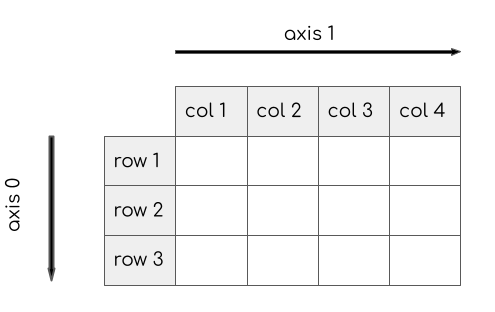from geopy import distance

def calculate_distance(row):
city_coordinates = (row['lat'], row['lng'])
return distance.geodesic(city_coordinates, home_city_coordinates).km

result = country_df.apply(calculate_distance, axis=1)
print(result)

We can add these results to the dataframe by simply assigning the result to a new column.

country_df['distance'] = result
print(country_df)

We are done with our analysis and ready to save the results. We can further filter the results to only certain columns.

filtered = country_df[['city_ascii','distance']]
print(filtered)

Let’s rename the city_ascii column to give it a more readable name.

filtered = filtered.rename(columns = {'city_ascii': 'city'})
print(filtered)

Now that we have added filtered the original data and computed the distance for all cities, we can save the resulting dataframe to a file. Similar to read methods, Pandas have several write methods, such as to_csv(), to_excel() etc.

Here we will use the to_csv() method to write a CSV file. Pandas assigns an index column (unique integer values) to a dataframe by default. We specify index=False so that this index is not added to our output.

output_filename = 'cities_distance_pandas.csv'
output_dir = 'output'
output_path = os.path.join(output_dir, output_filename)
filtered.to_csv(output_path, index=False)
print('Successfully written output file at {}'.format(output_path))

## Exercise

You will notice that the output file contains a row with the home_city as well. Modify the filtered dataframe to remove this row and write it to a file.

Hint: Use the Boolean filtering method we learnt earlier to select rows that do not match the home_city.

# Working with GeopandasGeoPandas extends the Pandas library to enable spatial operations. It provides new data types such as GeoDataFrame and GeoSeries which are subclasses of Pandas DataFrame and Series and enables efficient vector data processing in Python.

GeoPandas make use of many other widely used spatial libraries - but it provides an interface similar to Pandas that make it intuitive to use it with spatial analysis. GeoPandas is built on top of the following libraries that allow it to be spatially aware.

• Shapely for geometric operations (i.e. buffer, intersections etc.)
• PyProj for working with projections
• Fiona for file input and output, which itself is based on the widely used GDAL/OGR library

We will carry out a geoprocessing task that shows various features of this library and show how to do geo data processing in Python. The task is to take a roads data layer from OpenStreetMap and compute the total length of National Highways for each district in a state. The problem is described in detail in my Advanced QGIS course and show the steps needed to perform this analysis in QGIS. We will replicate this example in Python.By convention, geopandas is commonly imported as gpd

import geopandas as gpd

import os
data_pkg_path = 'data'
filename = 'karnataka.gpkg'
path = os.path.join(data_pkg_path, filename)

GeoPandas has a read_file() method that is able to open a wide variety of vector datasets, including zip files. Here we will open the GeoPackage karnataka.gpkg and read a layer called karnataka_major_roads. The result of the read method is a GeoDataFrame.

roads_gdf = gpd.read_file(path, layer='karnataka_major_roads')
print(roads_gdf.info())

A GeoDataFrame contains a special column called geometry. All spatial operations on the GeoDataFrame are applied to the geomety column. The geometry column can be accessed using the geometry attribute.

print(roads_gdf.geometry)

## Filtering Data

One can use the standard Pandas filtering methods to select a subset of the GeoDataFrame. In addition, GeoPandas also provide way to subset the data based on a bounding box with the cx[] indexer.

For our analysis, we need to apply a filter to extract only the road segments where the ref attribute starts with ‘NH’ - indicating a national highway. We can apply boolean filtering using Panda’s str.match() method with a regular expression.

filtered = roads_gdf[roads_gdf['ref'].str.match('^NH') == True]
print(filtered.head())

## Working with Projections

Dealing with projetions is a key aspect of working with spatial data. GeoPandas uses the pyproj library to assign and manage projections. Each GeoDataFrame as a crs attribute that contains the projection info. Our source dataset is in EPSG:4326 WGS84 CRS.

print(filtered.crs)

Since our task is to compute line lengths, we need to use a Projected CRS. We can use the to_crs() method to reproject the GeoDataFrame.

roads_reprojected = filtered.to_crs('EPSG:32643')
print(roads_reprojected.crs)

Now that the layer has been reprojected, we can calculate the length of each geometry using the length attribute. The result would be in meters. We can add the line lengths in a new column named length.

roads_reprojected['length'] = roads_reprojected['geometry'].length

We can apply statistical operations on a DataFrame columns. Here we can compute the total length of national highways in the state by calling the sum() method.

total_length = roads_reprojected['length'].sum()
print('Total length of national highways in the state is {} KM'.format(total_length/1000))

## Performing Spatial joins

There are two ways to combine datasets in geopandas – table joins and spatial joins. For our task, we need information about which district each road segments belongs to. This can be achived using another spatial layer for the districts and doing a spatial join to transfer the attributes of the district layer to the matching road segment.

The karnataka.gpkg contains a layer called karnataka_districts with the district boundaries and names.

districts_gdf = gpd.read_file(path, layer='karnataka_districts')
print(districts_gdf.head())

Before joining this layer to the roads, we must reproject it to match the CRS of the roads layer.

districts_reprojected = districts_gdf.to_crs('EPSG:32643')

A spatial join is performed using the sjoin() method. It takes 2 core arguments.

• op: The spatial predicate to decdie which objects to join. Options are intersects, within and contains.
• how: The type of join to perform. Options are left, right and inner.

For our task, we can do a left join and add attributes of the district that intersect the road.

joined = gpd.sjoin(roads_reprojected, districts_reprojected, how='left', op='intersects')
print(joined.head())

## Group Statistics

The resulting geodataframe now has the matching column from the intersecting district feature. We can now sum the length of the roads and group them by districts. This type of Group Statistics is performed using Panda’s group_by() method.

results = joined.groupby('DISTRICT')['length'].sum()/1000
print(results)

The result of the group_by() method is a Pandas Series. It can be saved to a CSV file using the to_csv() method.

output_filename = 'national_highways_by_districts.csv'
output_dir = 'output'
output_path = os.path.join(output_dir, output_filename)
results.to_csv(output_path)
print('Successfully written output file at {}'.format(output_path))

## Exercise

Before writing the output to the file, round the distance numbers to a whole number.

Open the notebook named 13_creating_spatial_data.ipynb.

# Creating Spatial Data

A common operation in spatial analysis is to take non-spatial data, such as CSV files, and creating a spatial dataset from it using coordinate information contained in the file. GeoPandas provides a convenient way to take data from a delimited-text file, create geometry and write the results as a spatial dataset.

We will read a tab-delimited file of places, filter it to a feature class, create a GeoDataFrame and export it as a GeoPackage file.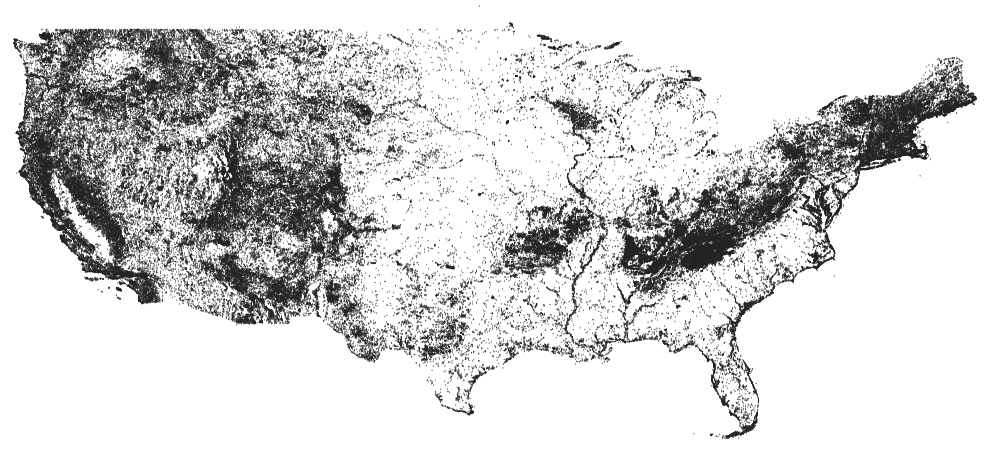import os
import pandas as pd
import geopandas as gpd
data_pkg_path = 'data/geonames/'
filename = 'US.txt'
path = os.path.join(data_pkg_path, filename)

The source data comes from GeoNames - a free and open database of geographic names of the world. It is a huge database containing millions of records per country. The data is distributed as country-level text files in a tab-delimited format. The files do not contain a header row with column names, so we need to specify them when reading the data. The data format is described in detail on the Data Export page.

We specify the separator as \t (tab) as an argument to the read_csv() method. Note that the file for USA has more than 2M records.

column_names = [
'geonameid', 'name', 'asciiname', 'alternatenames',
'latitude', 'longitude', 'feature class', 'feature code',
'dem', 'timezone', 'modification date'
]

print(df.info())

## Filtering Data

The input data as a column feature_class categorizing the place into 9 feature classes. We can select all rows with the value T with the category mountain,hill,rock…

mountains = df[df['feature class']=='T']
print(mountains.head()[['name', 'latitude', 'longitude', 'dem','feature class']])

## Creating Geometries

GeoPandas has a conveinent function points_from_xy() that creates a Geometry column from X and Y coordinates. We can then take a Pandas dataframe and create a GeoDataFrame by specifying a CRS and the geometry column.

geometry = gpd.points_from_xy(mountains.longitude, mountains.latitude)
gdf = gpd.GeoDataFrame(mountains, crs='EPSG:4326', geometry=geometry)
print(gdf.info())

## Writing Files

We can write the resulting GeoDataFrame to any of the supported vector data format. Here we are writing it as a new GeoPackage file.

You can open the resulting geopackage in a GIS and view the data.

output_dir = 'output'
output_filename = 'mountains.gpkg'
output_path = os.path.join(output_dir, output_filename)

gdf.to_file(driver='GPKG', filename=output_path, encoding='utf-8')
print('Successfully written output file at {}'.format(output_path))

## Exercise

The data package contains multiple geonames text files from different countries in the geonames/ folder. Write code to read all the files, merge them and extract the mountain features to a single geopackage.

• Hint1: Use the os.listdir() method to get all files in a directory.
• Hint2: Use the Pandas method concat() to merge multiple dataframes.
import os
import pandas as pd
import geopandas as gpd

data_pkg_path = 'data/geonames/'
files = os.listdir(data_pkg_path)

filepaths = []
for file in files:
filepaths.append(os.path.join(data_pkg_path, file))
print(filepaths)

# Iterate over the files, read them using pandas and create a list of dataframes.
# You can then use pd.concat() function to merge them

Open the notebook named 14_introduction_to_numpy.ipynb.

# Introduction to NumPyNumPy (Numerical Python) is an important Python library for scientific computation. Libraries such as Pandas and GeoPandas are built on top of NumPy.

It provides a fast and efficient ways to work with Arrays. In the domain of spatial data analysis, it plays a critical role in working with Raster data - such as satellite imagery, aerial photos, elevation data etc. Since the underlying structure of raster data is a 2D array for each band - learning NumPy is critical in processing raster data using Python.

By convention, numpy is commonly imported as np

import numpy as np

## Arrays

The array object in NumPy is called ndarray. It provides a lot of supporting functions that make working with arrays fast and easy. Arrays may seem like Python Lists, but ndarray is upto 50x faster in mathematical operations. You can create an array using the array() method. As you can see, the rsulting object is of type numpy.ndarray

a = np.array([1, 2, 3, 4])
print(type(a))

Arrays can have any dimensions. We can create a 2D array like below. ndarray objects have the property ndim that stores the number of array dimensions. You can also check the array size using the shape property.

b = np.array([[1, 2, 4], [3, 4, 5]])
print(b)
print(b.ndim)
print(b.shape)

You can access elements of arrays like Python lists using [] notation.

print(b)
print(b)

## Array Operations

Mathematical operations on numpy arrays are easy and fast. NumPy as many built-in functions for common operations.

print(np.sum(b))

You can also use the functions operations on arrays.

c = np.array([[2, 2, 2], [2, 2, 2]])
print(np.divide(b, c))

If the objects are numpy objects, you can use the Python operators as well

print(b/c)

You can also combine array and scalar objects. The scalar operation is applied to each item in the array.

print(b)
print(b*2)
print(b/2)

An important concept in NumPy is the Array Axes. Similar to the pandas library, In a 2D array, Axis 0 is the direction of rows and Axis 1 is the direction of columns. The diagram below show the directions.Let’s see how we can apply a function on a specific axis. Here when we apply sum function on axis-0 of a 2D array, it gives us a 1D-array with values summed across rows.

print(b)
row_sum = b.sum(axis=0)
print(row_sum)

## Exercise

Sum the array b along Axis-1. What do you think will be the result?

import numpy as np

b = np.array([[1, 2, 4], [3, 4, 5]])
print(b)

Open the notebook named 15_working_with_rasterio.ipynb.

# Working with RasterIO

RasterIO is a modern library to work with geospatial data in a gridded format. It excels at providing an easy way to read/write raster data and access individual bands and pixels as numpy arrays.

RasterIO is built on top of the popular GDAL (Geospatial Data Abstraction Library). GDAL is written in C++ so the Python API provided by GDAL is not very intuitive for Python users. RaserIO aims to make it easy for Python users to use the underlying GDAL library in an intuitive way.

In this section, we will take 4 individual SRTM tiles around the Mt. Everest region and merge them to a single GeoTiff using RasterIO.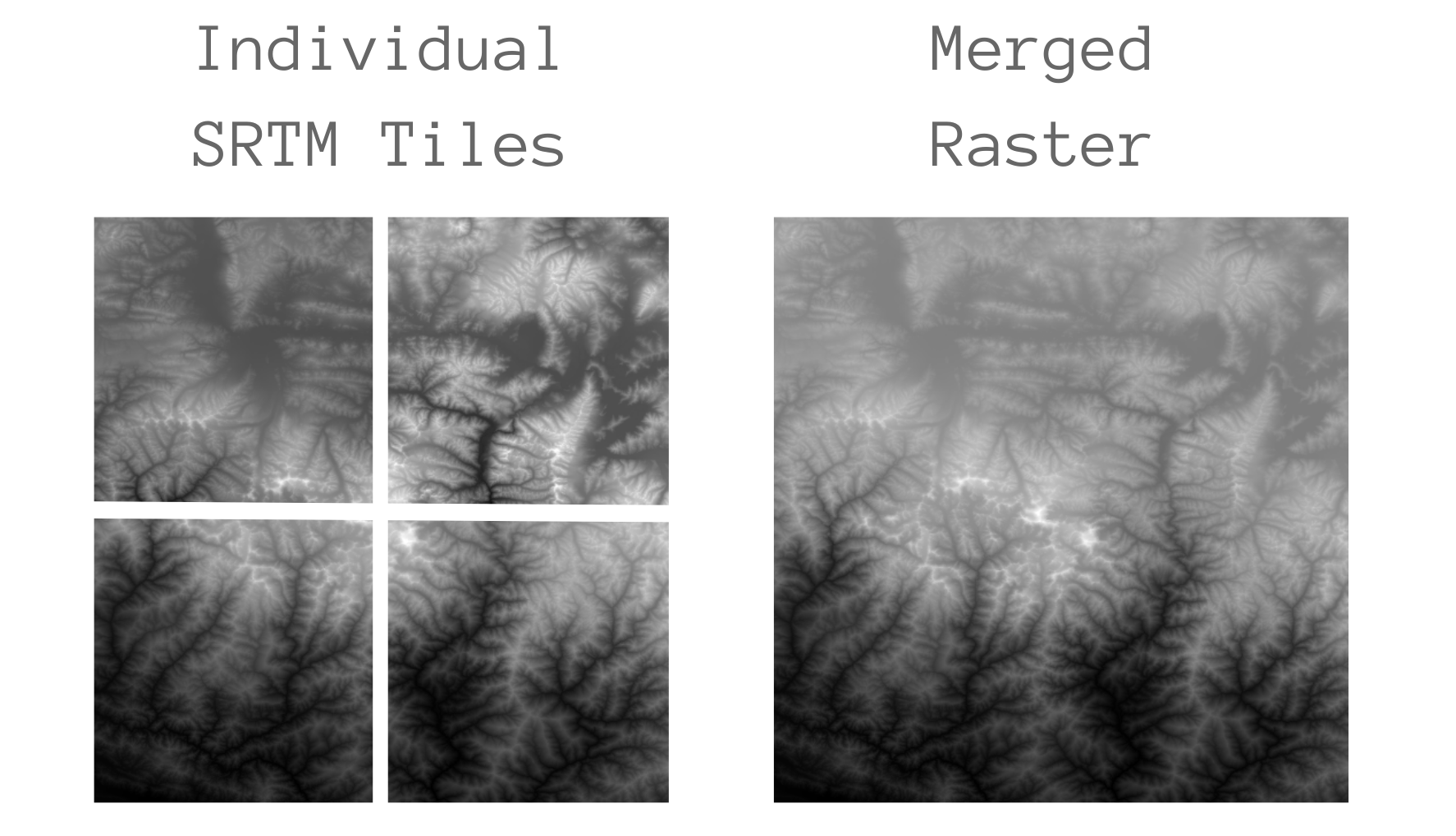import rasterio
import os
data_pkg_path = 'data'
srtm_dir = 'srtm'
filename = 'N28E087.hgt'
path = os.path.join(data_pkg_path, srtm_dir, filename)

RasterIO can read any raster format supported by the GDAL library. We can call the open() method with the file path of the raster. The resulting dataset behaves much like Python’s File object.

dataset = rasterio.open(path)

You can check information about the raster using the meta attribute.

An important property is the dataset transform. The transform contains the pixel resolution of the dataset and the row and column coordinates of the upper left corner of the dataset.

metadata = dataset.meta
metadata

To read the pixel values, we need to call the read() method by passing it a band’s index number. Following the GDAL convention, bands are indexed from 1. Since our dataset contain just 1-band, we can read it as follows.

band1 = dataset.read(1)
print(band1)

Finally, when we are done with the dataset, we must close it. It is especially important when writing a dataset.

dataset.close()

## Merging Datasets

Let’s see how we can read the 4 individual tiles and mosaic them together. RasterIO provides multiple sub-modules for various raster operations. We can use the rasterio.merge module to carry out this operation.

We first find all the individual files in the directory using the os.listdir() function.

srtm_path = os.path.join(data_pkg_path, 'srtm')
all_files = os.listdir(srtm_path)
print(python-foundation-output/all_files)

The rasterio.merge module has a merge() method that takes a list of datasets and returns the merged dataset. So we create an empty list, open each of the files and append it to the list.

dataset_list = []
for file in all_files:
path = os.path.join(srtm_path, file)
dataset_list.append(rasterio.open(path))
print(dataset_list)

We can pass on the list of tile dataset to the merge method, which will return us the merged data and a new transform which contains the updated extent of the merged raster.

from rasterio import merge
merged_result = merge.merge(dataset_list)
print(merged_result)

We save the data and the transform to separate variables.

merged_data = merged_result
merged_transform = merged_result

Verify that the resulting array shape the sum of individual rasters

print(merged_data.shape)

## Writing Raster Data

Similar to regular Python files, to create a new file, we can open the output file in the write mode. RasterIO provides a write() method that we can use to write individual bands.

output_filename = 'merged.tif'
output_dir = 'output'
output_path = os.path.join(output_dir, output_filename)

We need to specify many metadata parameters to initialize the output dataset. Some of these parameter values can be directly copied from the input files, such as crs, dtype, nodata etc. , while others can be obtained from the merged dataset, such as height and width.

Remember to call the close() method which will finalize the file and write the data to disk.

new_dataset = rasterio.open(output_path, 'w',
driver='GTiff',
height=merged_data.shape,
width=merged_data.shape,
count=1,
nodata=-32768.0,
dtype=merged_data.dtype,
crs='+proj=latlong',
transform=merged_transform)
new_dataset.write(merged_data)
new_dataset.close()
print('Successfully written output file at {}'.format(output_path))

## Exercise

The merged array represents elevation values. The extent of the tiles cover Mt. Everest. Read the resulting raster and find the maximum elevation value contained in it.

import rasterio
import os
import numpy as np

output_filename = 'merged.tif'
output_dir = 'output'
output_path = os.path.join(output_dir, output_filename)

# Read the output file as a NumPy array and find the maximum value

# Writing Standalone Python Scripts

So far we have used Jupyter Notebooks to write and execute Python code. A notebook is a great choice to interactively explore, visualize and document workflows. But they are not suited for writing scripts for automation. If you have tasks that are long running or want to execute certain tasks on a schedule, you have to write scripts in a standalone .py file and run it from a Terminal or Console.

## Get a Text Editor

Any kind of software development requires a good text editor. If you already have a favorite text editor or an IDE (Integrated Development Environment), you may use it for this course. Otherwise, each platform offers a wide variety of free or paid options for text editors. Choose the one that fits your needs.

Below are my recommendations editors that are simple to use for beginners.

• Windows: Notepad++ is a good free editor for windows. Download and install the Notepad++ editor. Tip: Before writing Python code in Notepad+++, make sure to go to Settings → Preferences → Tab Settings and enable Replace by space. Python is very sensitive about whitespace and this setting will ensure tabs and spaces are treated properly.
• Mac: TextMate is an open-source editor for Mac that is currently available for free.

## Writing a Script

Copy the following code and paste it in your text editor. Browse to the data package directory and save the file as get_distance.py. Make sure that there is no .txt extension at the end.

from geopy import distance

san_francisco = (37.7749, -122.4194)
new_york = (40.661, -73.944)

ellipsoid_distance = distance.geodesic(san_francisco, new_york, ellipsoid='WGS-84').km

print('Source Coordinates: {},{}'.format(san_francisco, san_francisco))
print('Destination Coordinates: {},{}'.format(san_francisco, san_francisco))
print('Elliosoid Distance: {} km'.format(ellipsoid_distance))

## Executing a Script

### Windows

1. Launch the Anaconda Navigator program. Select the python_foundation environment and launch the CMD.exe Prompt program.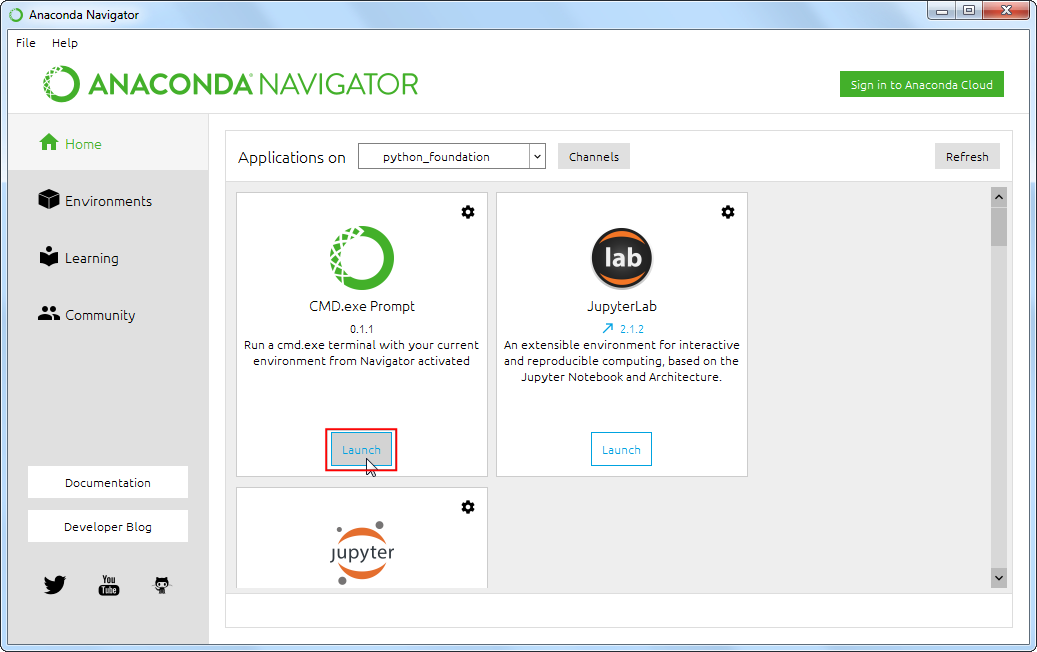1. Navigate to the directory containing the script using the cd command.
cd Downloads\python_foundation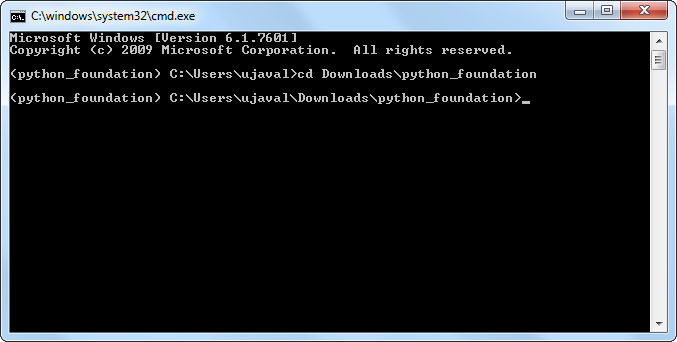1. Run the script using the python command. The script will run and print the distance.
python get_distance.py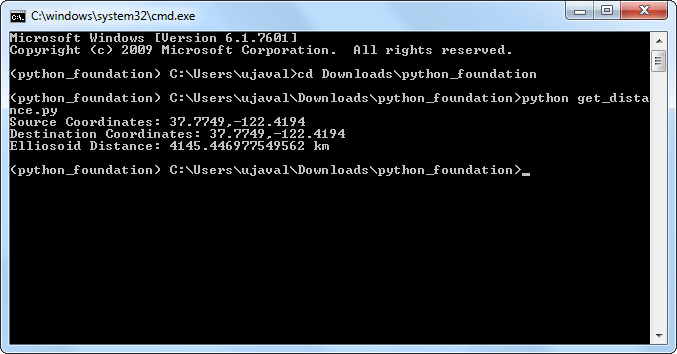### Mac and Linux

1. Open a Terminal Window.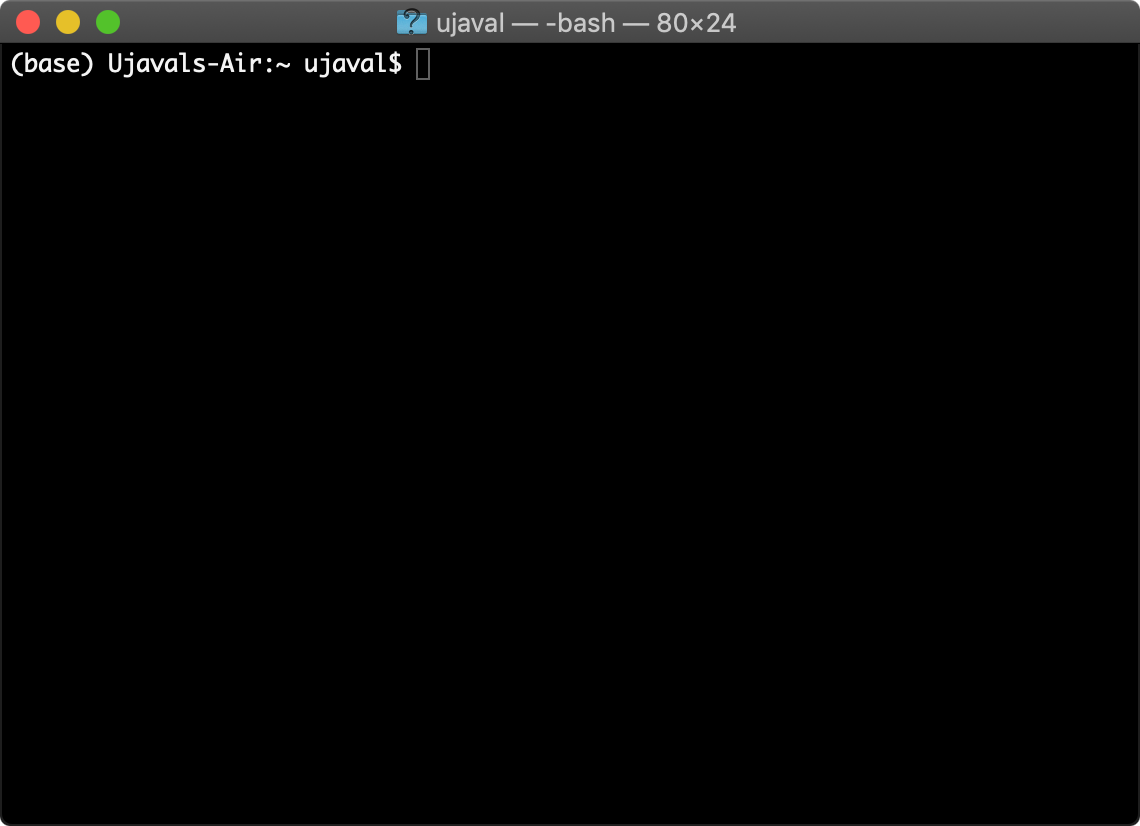1. Switch to the correct conda environment.
conda activate python_foundation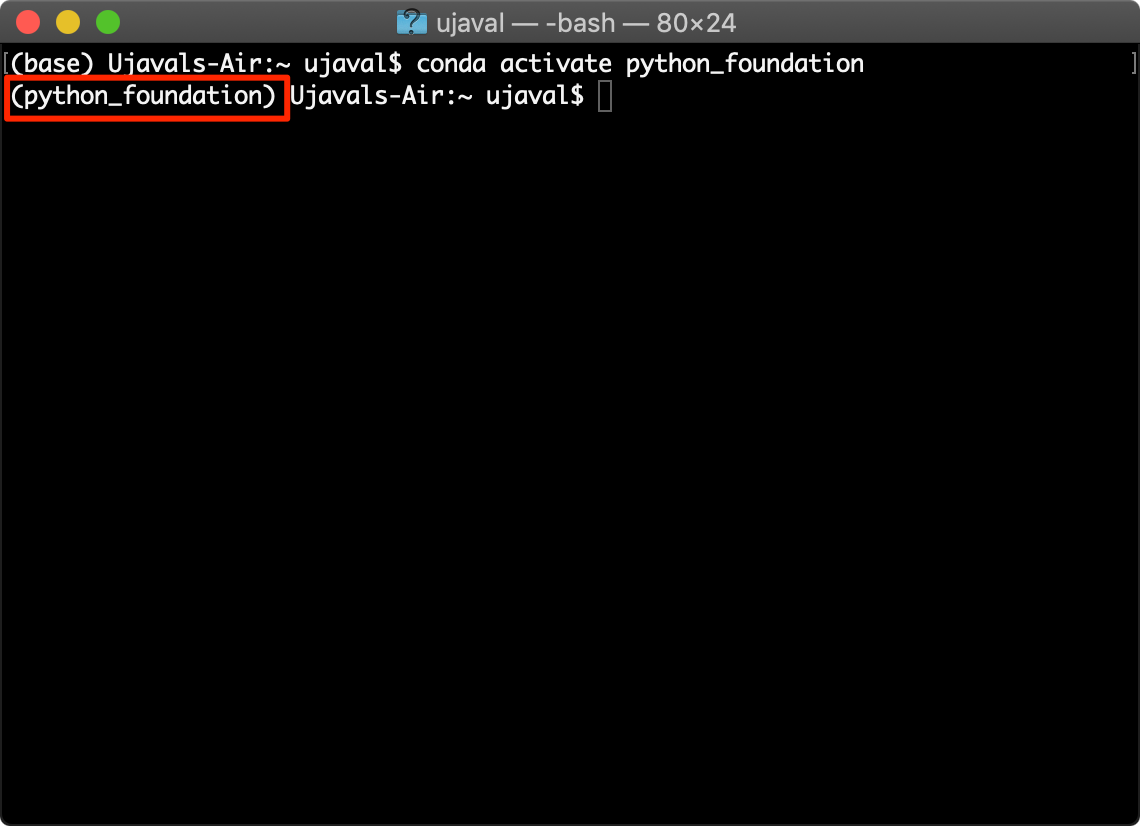1. Navigate to the directory containing the script using the cd command.
cd Downloads/python_foundation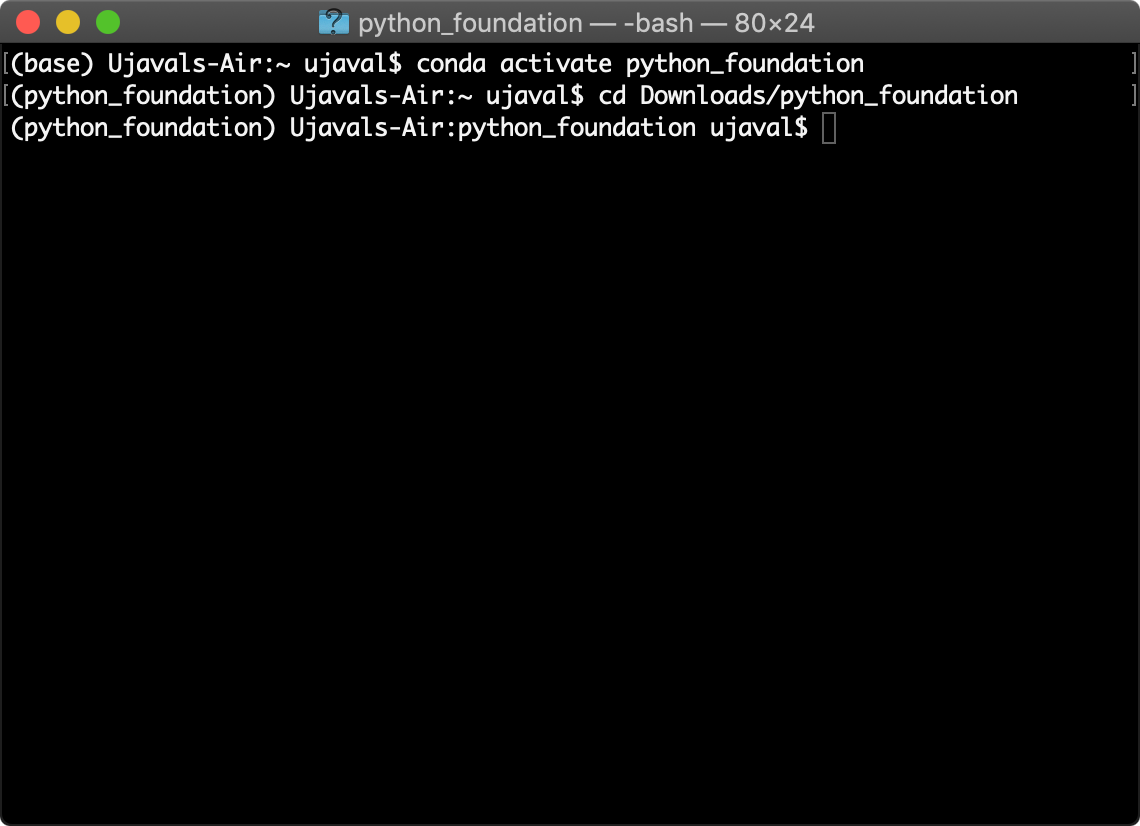1. Run the script using the python command. The script will run and print the distance.

If you have multiple python installations on your system, you will have to pick the right Python binary. If the command fails, try python3.7 instead of just python in the command below. The script will run and print the distance.

python get_distance.py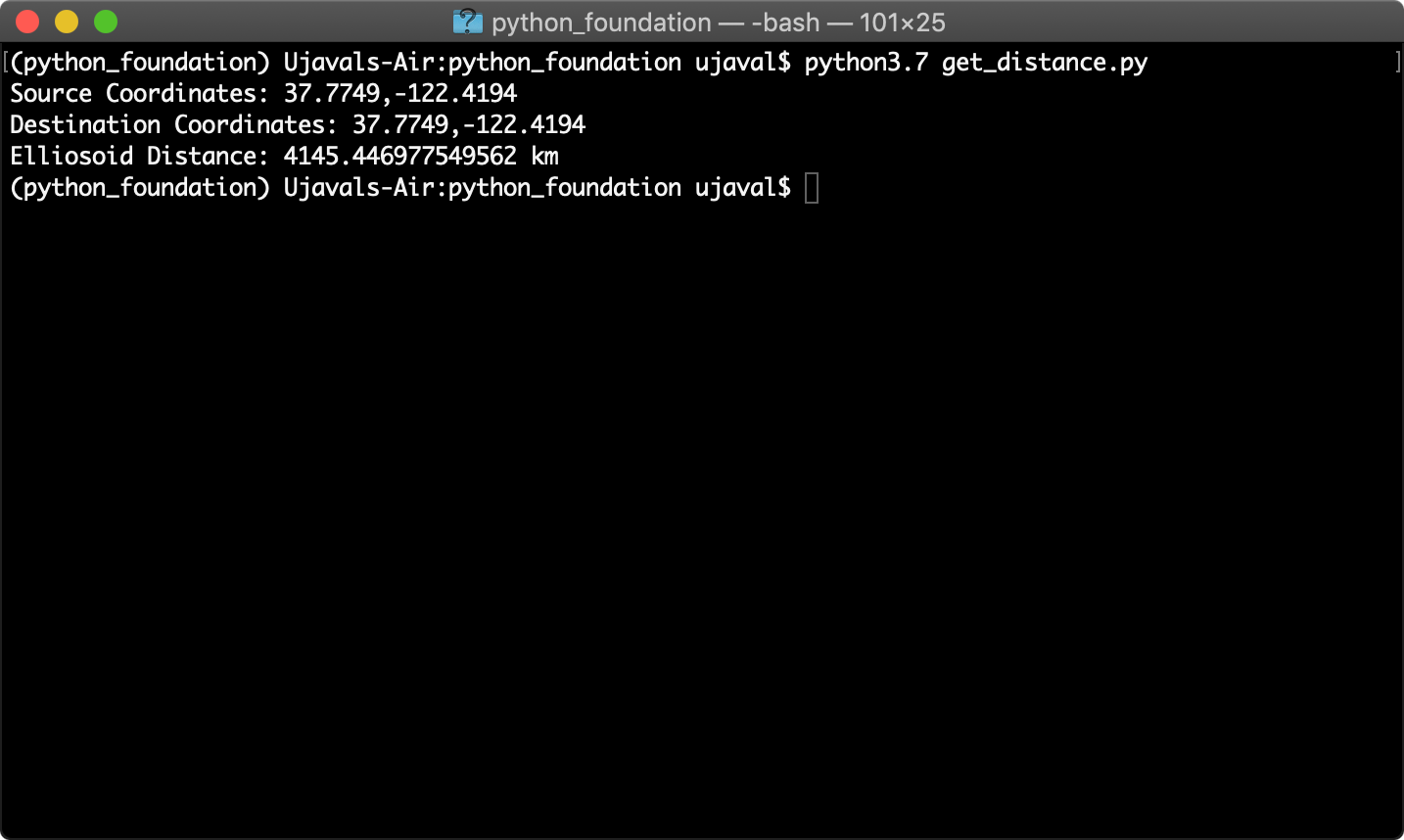# What next?

To achieve mastery in programming, you need to practice writing code and solve many diverse type of problems. I strongly recommend picking up a project and implementing it in Python to improve your skills. You can also take other courses to learn aspects of Python and spatial data processing.

## Do a Project

I have some recommendation for projects that are a good fit for beginners, but are still challenging to help you become proficient. You can try to work on as many of the following problems as you like.

• Geocoding: Find list of addresses and geocode them to create a point layer. Here’s a good overview of geocoding using geopy
• Network Analysis: Use the osmnx package to do network analysis with OpenStreetMap data and Python. Start by looking at examples
• Visualization and Interactive Mapping: Use the plotting library geoplot or mapping library folium to create an interactive map. See this example
• Geo-processing: I have several intermediate level geoprocessing tutorials that use QGIS. You can take any of the following tutorials and implement it using libraries such as geopandas and rasterio.

You may want to check out this list of useful python geo-spatial libraries for your project.

## Keep Learning

Here are some recommendations for courses you could take after you have completed this course.

# Supplement

## Creating Maps with GeoPandas

GeoPandas comes with built-in functions for visualizing geospatial data and creating maps. It uses the very powerful matplotlib library to do the plotting. If you are not familiar with matplotlib, check out this introductory tutorial.

This notebook shows how we can create visualizaion using the datasets from the Working with GeoPandas exercise.

import geopandas as gpd
import os
data_pkg_path = 'data'
filename = 'karnataka.gpkg'
path = os.path.join(data_pkg_path, filename)
national_highways = roads[roads['ref'].str.match('^NH') == True]

### Matplotlib Basics

Before we start using matplotlib inside a Jupyter notebook, it is useful to set the matplotlib backend to inline. This setting makes the matplotlib graphs included in your notebook, next to the code. Learn more about different plotting backends.

We use the magic function %matplotlib to achieve this.

%matplotlib inline
import matplotlib.pyplot as plt

It is important to understand the 2 matplotlib objects

• Figure: This is the main container of the plot. A figure can contain multiple plots inside it
• Axes: Axes refers to an individual plot or graph. A figure contains 1 or more axes.

We create a figure and a single subplot. Specifying 1 row and 1 column for the subplots() function create a figure and an axes within it. Even if we have a single plot in the figure, it is useful to use tthis logic of intialization so it is consistent across different scripts.

fig, ax = plt.subplots(1, 1)
fig.set_size_inches(5,5)
plt.show()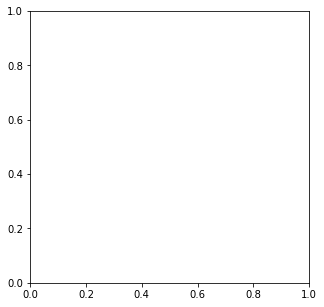First, let’s learn how to plot a single point using matplotlib. Let’s say we want to display a point at the coordinate (0.5, 0.5).

point = (0.5, 0.5)

We display the point using the plot() function. The plot() function expects at least 2 arguments, first one being one or more x coordinates and the second one being one or more y coordinates. Remember that once a plot is displayed using plt.show(), it displays the plot and empties the figure. So you’ll have to create it again.

fig, ax = plt.subplots(1, 1)
fig.set_size_inches(5,5)
ax.plot(point, point, color='green', marker='o')
plt.show()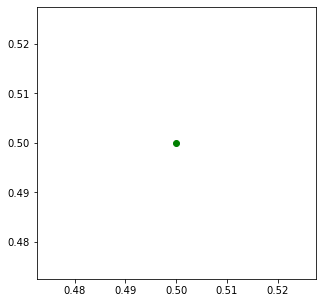One problematic area for plotting geospatial data using matplotlib is that geospatial data is typically represented as a list of x and y coordinates. But to plot it, we require 2 separate lists or x and y coordinates. Here we can use the zip() function to create list of x and y coordinates.

points = [(0.1, 0.5), (0.5, 0.5), (0.9, 0.5)]
x, y = zip(*points)
print(x)
print(y)
(0.1, 0.5, 0.9)
(0.5, 0.5, 0.5)

Now these can be plotted using the plot() function. We can set linestyle=None so it displays the points but doesn’t connect them. You can also use ax.scatter(x, y) to create a scatter plot with the same result.

fig, ax = plt.subplots(1, 1)
fig.set_size_inches(5,5)
ax.plot(x, y, color='green', marker='o', linestyle='none')
plt.show()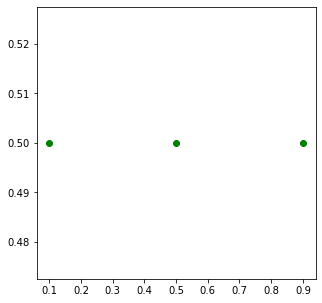GeoPandas provides a convenient plot() function for GeoDataFrame and GeoSeries objects. It takes care of doing the coordinate transformations so that it can be plotted using matplotlib. Calling .plot() on a GeoDataFrame returns a matplotlib axes instance. Any options one can pass to matplotlib as seen before can be used with the GeoPandas plot() function. Learn more about mapping and plotting using GeoPandas.

We will now see different examples of creating maps.

### Rendering Map Layouts

We can now work on creating a figure with multiple axes - each with a different rendering on a map layer. The subplots() function creates one or more plots within the figure. You can design a map layout with multiple rows/columns. In the code below, we create a map with 1 row and 3 columns. Using the set_size_inches() function, we set the size of the map to 15in x 7in.

fig, axes = plt.subplots(1, 3)
fig.set_size_inches(15,7)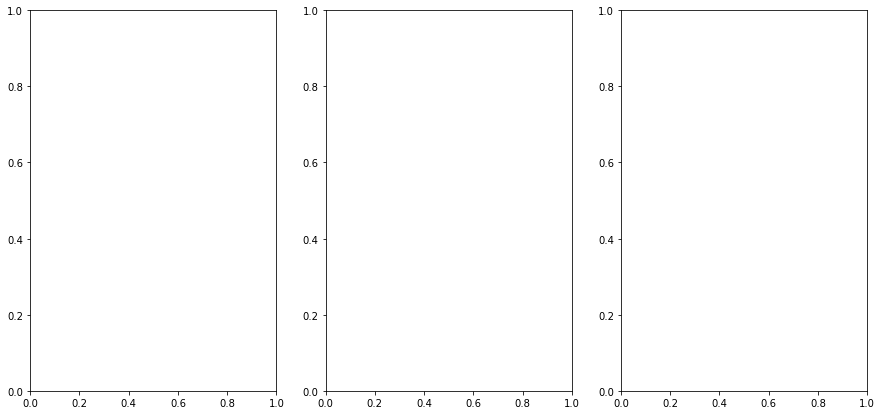The subplots() function returns 2 items. The figure and a tuple with all the axes within the figure. As we have 3 axes, we unpack them into separate variables

ax0, ax1, ax2 = axes

GeoDataFrame objects have a plot() method that uses pyplot and creates a plot. We supply the ax object to the function so the resulting plot is displayed in the Axes created previously. Here we add the districts polygon layer into the ax0 object - which refers to the first subplot.

districts.plot(ax=ax0, linewidth=1, facecolor='none', edgecolor='#252525')
fig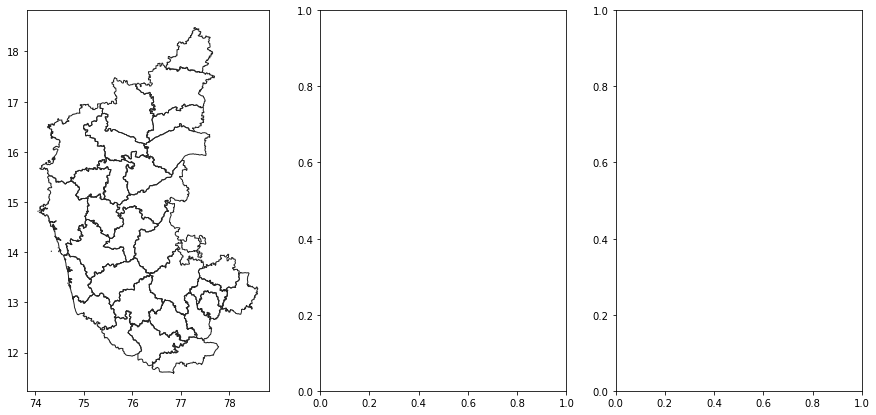<Figure size 432x288 with 0 Axes>

Similarly, we add the roads layer to the second axes.

roads.plot(ax=ax1, linewidth=0.4, color='#2b8cbe')
fig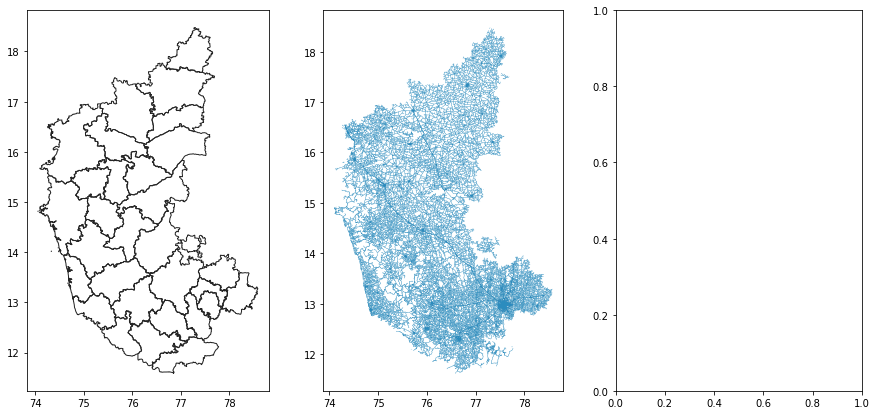<Figure size 432x288 with 0 Axes>

Lastly, we add the national_highways layer to the third axes.

national_highways.plot(ax=ax2, linewidth=1, color='#de2d26')
fig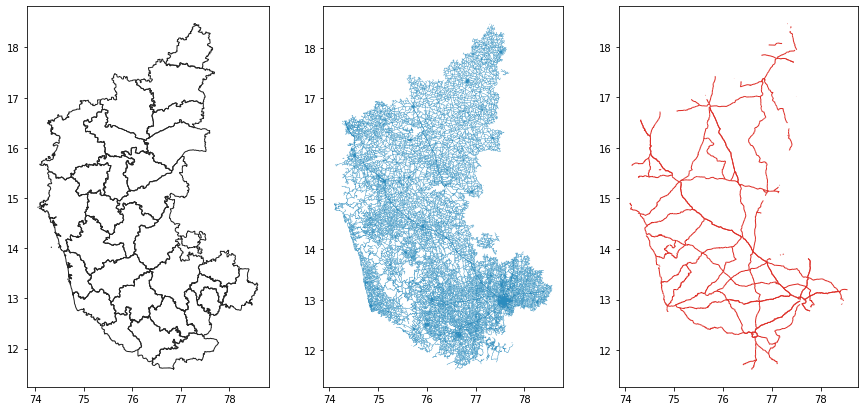<Figure size 432x288 with 0 Axes>

We can turn off the coordinates display on the X-axis and Y-axis using plt.axis('off'). It is useful to set a title to each map. The set_title() function adds the title to the approproate axes. We specify a negative y parameter to place the title at the bottom of the map instead of top.

ax0.axis('off')
ax0.set_title('Karnataka Districts', y=-0.1)
ax1.axis('off')
ax2.axis('off')
ax2.set_title('Karnataka National Highways', y=-0.1)
fig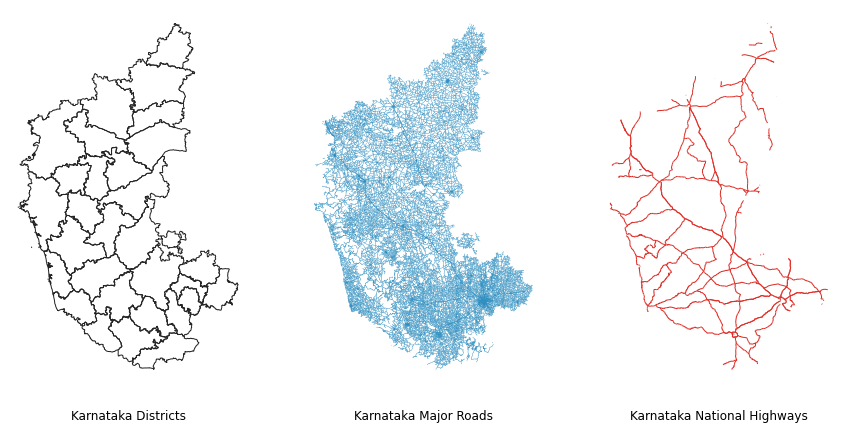Now that our map is ready, we can save the map to the computer using the savefig() function.

output_filename = 'map_layout.png'
output_dir = 'output'
output_path = os.path.join(output_dir, output_filename)

if not os.path.exists(output_dir):
os.mkdir(output_dir)

fig.savefig(output_path, dpi=300)

### Creating A Map with Multiple Layers

If we want to display multiple layers, we simply create new plots on the same Axes. Here we create a figure with a single axes and add the districts,roads and national_highways layers to the same axes.

fig, ax = plt.subplots()
fig.set_size_inches(10,15)

plt.axis('off')

districts.plot(ax=ax, linewidth=1, facecolor='none', edgecolor='#252525')
national_highways.plot(ax=ax, linewidth=1, color='#de2d26')

output_filename = 'multiple_layers.png'
output_path = os.path.join(output_dir, output_filename)
plt.savefig(output_path, dpi=300)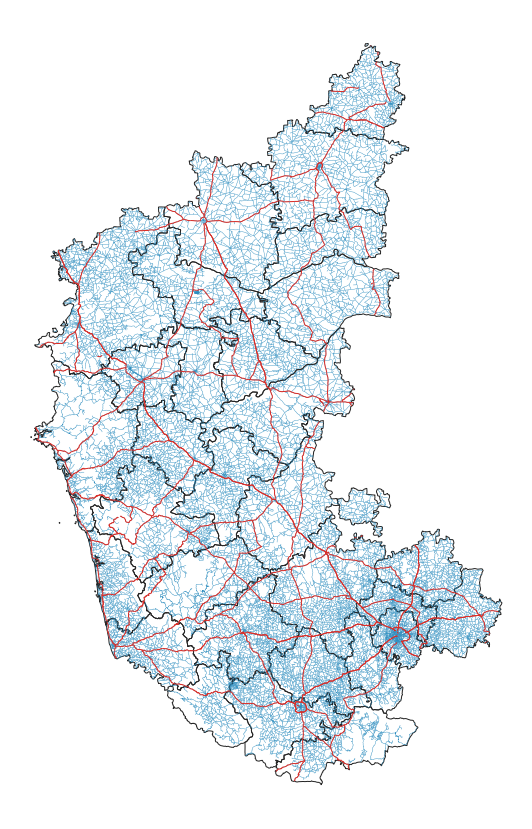### Labelling Features

We can also add labels to the maps, but that requires a bit of pre-processing. Let’s say we want to add a label for each of the distrit polygons. First, we need to decide the anchor position of the label. We can use representative_point() to get a point inside each polygon that best represents the geometry. It is similar to a centroid, but is guranteed to be inside the polygon. Below code creates a new field in the GeoDataFrame called label_position with the coordinates of the anchor point.

districts['label_position'] = districts['geometry'].apply(lambda x: x.representative_point().coords[:])
districts['label_position'] = [coords for coords in districts['label_position']]

Now we can use the annotate() function and iterate over each polygon to add labels with the name of the district from the DISTRICT column and place it at the coordinates from the label_position column.

fig, ax = plt.subplots(figsize=(10, 15))
plt.axis('off')

districts.plot(ax=ax, linewidth=1, facecolor='none', edgecolor='#252525')

for idx, row in districts.iterrows():
plt.annotate(text=row['DISTRICT'], xy=row['label_position'], horizontalalignment='center')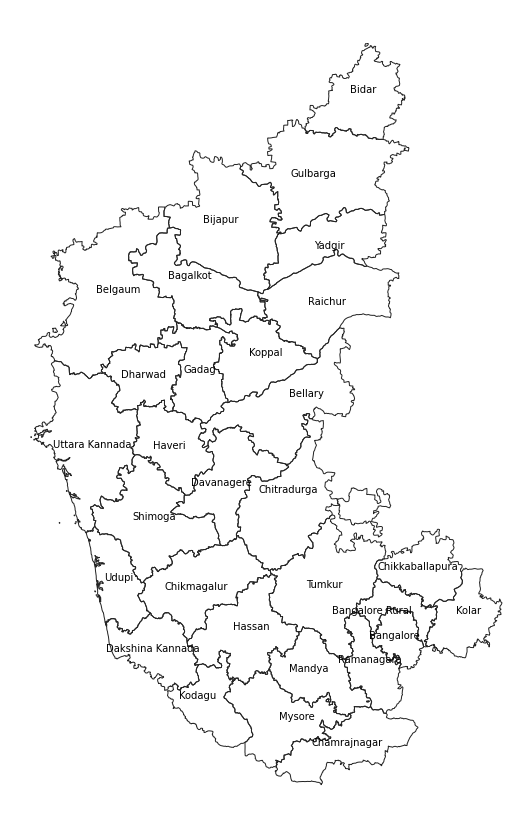View the Notebook ↗

## Working with Xarray

Xarray is an evolution of rasterio and is inspired by libraries like pandas to work with raster datasets. It is particularly suited for working with multi-dimensional time-series raster datasets. It also integrates tightly with dask that allows one to scale raster data processing using parallel computing.

rioxarray is an extension of xarray that makes it easy to work with geospatial rasters. You can install the rioxarray package from the conda-forge channel.

This notebook shows how we can replicate the analysis from the Working with RasterIO exercise and also covers raster data visualization using matplotlib.

### XArray and rioxarray Basics

We start by reading a single SRTM tile containing elevation values.

import os
data_pkg_path = 'data'
srtm_dir = 'srtm'
filename = 'N28E087.hgt'
path = os.path.join(data_pkg_path, srtm_dir, filename)

By convention, rioxarray is imported as rxr

import rioxarray as rxr

The open_rasterio() method is able to read any data source supported by rasterio library.

rds = rxr.open_rasterio(path)

The result is a xarray.DataArray object.

type(rds)
xarray.core.dataarray.DataArray

You can access the pixel values using the values property which returns the array’s data as a numpy array.

rds.values
array([[[5217, 5211, 5208, ..., 5097, 5098, 5089],
[5206, 5201, 5200, ..., 5080, 5075, 5069],
[5199, 5194, 5191, ..., 5063, 5055, 5048],
...,
[5347, 5345, 5343, ..., 5747, 5750, 5757],
[5338, 5338, 5336, ..., 5737, 5740, 5747],
[5332, 5331, 5332, ..., 5734, 5736, 5744]]], dtype=int16)

A xarray.DataArray object also contains 1 or more coordinates. Each coordinate is a 1-dimensional array representing values along one of the data axes. In case of the 1-band SRTM elevation data, we have 3 coordinates - x, y and band.

rds.coords
Coordinates:
* band         (band) int64 1
* x            (x) float64 87.0 87.0 87.0 87.0 87.0 ... 88.0 88.0 88.0 88.0
* y            (y) float64 29.0 29.0 29.0 29.0 29.0 ... 28.0 28.0 28.0 28.0
spatial_ref  int64 0

A key feature of xarray is the ability to access slices of the dataset using index lookup methods. For example, we can slice the main dataset and get the data for Band1 using the sel() method.

band1 = rds.sel(band=1)

The raster metadata is stored in the rio accessor. This is enabled by the rioxarray library which provides geospatial functions on top of xarray.

print('CRS:', rds.rio.crs)
print('Resolution:', rds.rio.resolution())
print('Bounds:', rds.rio.bounds())
print('Width:', rds.rio.width)
print('Height:', rds.rio.height)
CRS: EPSG:4326
Resolution: (0.0002777777777777778, -0.0002777777777777778)
Bounds: (86.99986111111112, 27.999861111111112, 88.00013888888888, 29.000138888888888)
Width: 3601
Height: 3601

### Merging Rasters

Now that you understand the basic data structure of xarray and the &rio* extension, let’s use it to process some data. We will take 4 individual SRTM tiles and merge them to a single GeoTiff. You will note that rioxarray handles the CRS and transform much better - taking care of internal details and providing a simple API.

Remember to always import rioxarray even if you are using sub-modules. Importing rioxarray activates the rio accessor which is required for all operations.

import rioxarray as rxr
from rioxarray.merge import merge_arrays

Define input and output paths.

srtm_path = os.path.join(data_pkg_path, 'srtm')
all_files = os.listdir(srtm_path)
output_filename = 'merged.tif'
output_dir = 'output'
output_path = os.path.join(output_dir, output_filename)

Open each source file using open_rasterio() method and store the resulting datasets in a list.

datasets = []
for file in all_files:
path = os.path.join(srtm_path, file)
datasets.append(rxr.open_rasterio(path))

Use the merge_arrays() method from the rioxarray.merge module to merge the rasters.

merged = merge_arrays(datasets)

Finally, save the merged array to disk as a GeoTiff file.

merged.rio.to_raster(output_path)

### Visualizing Rasters using Matplotlib

xarray plotting functionality is built on top of the the popular matplotlib library.

%matplotlib inline
import matplotlib.pyplot as plt

You cna visualize any DataArray object by calling plot() method. Here we create a row of 4 plots and render each of the source SRTM rasters. We can use the cmap option to specify a color ramp. Here we are using the built-in Greys ramp. Appending **_r** gives us the inverted ramp with blacks representing lower elevation values.

fig, axes = plt.subplots(1, 4)
fig.set_size_inches(15,3)
plt.tight_layout()
for index, dataset in enumerate(datasets):
ax = axes[index]
dataset.plot(ax=ax, cmap='Greys_r')
ax.axis('off')
filename = all_files[index]
ax.set_title(filename)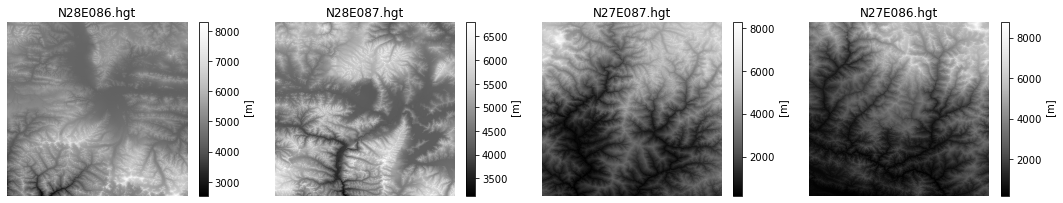Similarly, we can visualize the merged raster.

fig, ax = plt.subplots()
fig.set_size_inches(12, 10)
merged.plot(ax=ax, cmap='Greys_r')
ax.set_title('merged')
plt.show()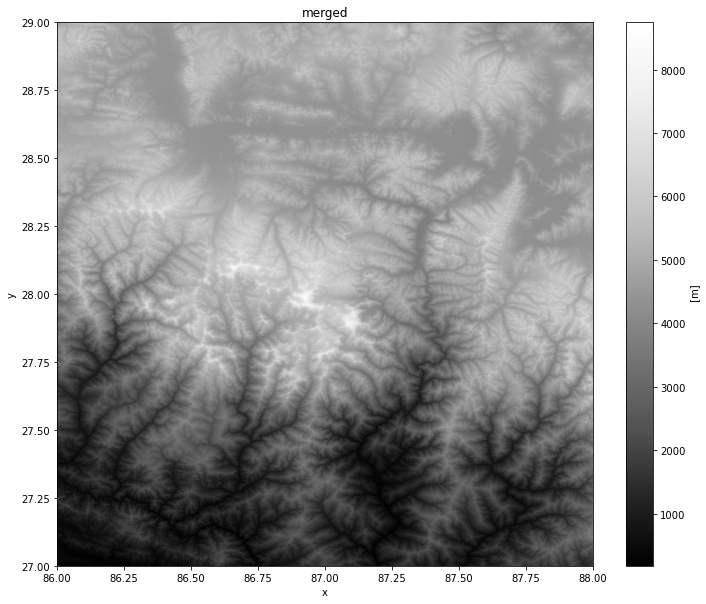View the Notebook ↗